# KR20140106436A - Battery state of charge tracking, equivalent circuit selection and benchmarking - Google Patents

Battery state of charge tracking, equivalent circuit selection and benchmarking Download PDF

## Info

Publication number
KR20140106436A
KR20140106436A KR1020140021544A KR20140021544A KR20140106436A KR 20140106436 A KR20140106436 A KR 20140106436A KR 1020140021544 A KR1020140021544 A KR 1020140021544A KR 20140021544 A KR20140021544 A KR 20140021544A KR 20140106436 A KR20140106436 A KR 20140106436A
Authority
KR
South Korea
Prior art keywords
battery
soc
voltage
estimated
ocv
Prior art date
Application number
KR1020140021544A
Other languages
Korean (ko)
Inventor
밸러쿠마르 밸러싱검
야코브 바르-샬롬
브라이언 프렌치
바래쓰 패티퍼티
크리쉬나 알. 패티퍼티
제임스 미캠
트레비스 윌리엄스
가피 비나드 어배리
황태식
Original Assignee
페어차일드 세미컨덕터 코포레이션
더 유니버시티 오브 코네티컷
Priority date (The priority date is an assumption and is not a legal conclusion. Google has not performed a legal analysis and makes no representation as to the accuracy of the date listed.)
Filing date
Publication date
Priority to US201361768472P priority Critical
Priority to US61/768,472 priority
Priority to US14/185,790 priority patent/US20140244193A1/en
Priority to US14/185,790 priority
Priority to US14/185,835 priority patent/US10664562B2/en
Priority to US14/185,835 priority
Application filed by 페어차일드 세미컨덕터 코포레이션, 더 유니버시티 오브 코네티컷 filed Critical 페어차일드 세미컨덕터 코포레이션
Publication of KR20140106436A publication Critical patent/KR20140106436A/en

• 244000171263 Ribes grossularia Species 0.000 claims description 153
• 206010057190 Respiratory tract infections Diseases 0.000 claims description 65
• 238000004422 calculation algorithm Methods 0.000 claims description 35
• 239000011159 matrix materials Substances 0.000 claims description 29
• 230000000875 corresponding Effects 0.000 claims description 24
• 238000003860 storage Methods 0.000 claims description 14
• 238000004364 calculation methods Methods 0.000 claims description 8
• 239000000446 fuels Substances 0.000 claims description 7
• 230000001186 cumulative Effects 0.000 claims 1
• 238000007600 charging Methods 0.000 abstract description 14
• 238000000034 methods Methods 0.000 description 53
• 238000010586 diagrams Methods 0.000 description 24
• 238000001914 filtration Methods 0.000 description 17
• 239000000203 mixtures Substances 0.000 description 15
• 238000005259 measurements Methods 0.000 description 11
• 230000000694 effects Effects 0.000 description 9
• 230000003044 adaptive Effects 0.000 description 8
• 239000003990 capacitor Substances 0.000 description 7
• 230000000284 resting Effects 0.000 description 7
• 230000005540 biological transmission Effects 0.000 description 5
• 230000002596 correlated Effects 0.000 description 5
• 238000009795 derivation Methods 0.000 description 5
• 238000005070 sampling Methods 0.000 description 5
• 229910052709 silver Inorganic materials 0.000 description 5
• 239000004332 silver Substances 0.000 description 5
• 230000032683 aging Effects 0.000 description 4
• 238000004458 analytical methods Methods 0.000 description 4
• 238000004590 computer program Methods 0.000 description 4
• 230000004927 fusion Effects 0.000 description 4
• 230000004048 modification Effects 0.000 description 4
• 238000006011 modification reactions Methods 0.000 description 4
• 230000001413 cellular Effects 0.000 description 3
• 230000001419 dependent Effects 0.000 description 3
• 239000006185 dispersions Substances 0.000 description 3
• 238000005562 fading Methods 0.000 description 3
• 230000001939 inductive effects Effects 0.000 description 3
• 230000003466 anti-cipated Effects 0.000 description 2
• 230000003190 augmentative Effects 0.000 description 2
• 238000007599 discharging Methods 0.000 description 2
• 238000009826 distribution Methods 0.000 description 2
• 230000014509 gene expression Effects 0.000 description 2
• 238000004519 manufacturing process Methods 0.000 description 2
• 230000003287 optical Effects 0.000 description 2
• 238000005457 optimization Methods 0.000 description 2
• 230000000737 periodic Effects 0.000 description 2
• 230000004044 response Effects 0.000 description 2
• 238000004088 simulation Methods 0.000 description 2
• 230000003068 static Effects 0.000 description 2
• 239000011901 water Substances 0.000 description 2
• 281000002255 Battery manufacturers companies 0.000 description 1
• 229920002574 CR-39 Polymers 0.000 description 1
• 239000004215 Carbon black (E152) Substances 0.000 description 1
• 280000409857 Coaxial Cable companies 0.000 description 1
• 241001023771 Escherichia virus TLS Species 0.000 description 1
• 280000867207 Lambda companies 0.000 description 1
• 280000091557 Plug and Play companies 0.000 description 1
• 281000056277 Storage Technology, Corp. companies 0.000 description 1
• 238000009825 accumulation Methods 0.000 description 1
• 238000009529 body temperature measurement Methods 0.000 description 1
• 239000000969 carriers Substances 0.000 description 1
• 238000006243 chemical reactions Methods 0.000 description 1
• 238000010277 constant-current charging Methods 0.000 description 1
• 125000004122 cyclic group Chemical group 0.000 description 1
• 238000005225 electronics Methods 0.000 description 1
• 238000004146 energy storage Methods 0.000 description 1
• 238000005516 engineering processes Methods 0.000 description 1
• 238000009472 formulation Methods 0.000 description 1
• 239000003365 glass fibers Substances 0.000 description 1
• 238000009499 grossing Methods 0.000 description 1
• 150000002430 hydrocarbons Chemical class 0.000 description 1
• 230000001788 irregular Effects 0.000 description 1
• 239000004973 liquid crystal related substances Substances 0.000 description 1
• 229910001416 lithium ion Inorganic materials 0.000 description 1
• 239000002245 particles Substances 0.000 description 1
• 230000002104 routine Effects 0.000 description 1
• 239000000126 substances Substances 0.000 description 1
• 238000006467 substitution reactions Methods 0.000 description 1
• 230000002123 temporal effects Effects 0.000 description 1
• 230000001052 transient Effects 0.000 description 1
• 201000003130 ventricular septal defect Diseases 0.000 description 1
• 230000002087 whitening Effects 0.000 description 1

## Images

•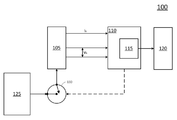•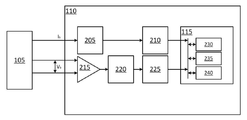•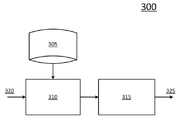•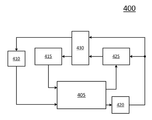•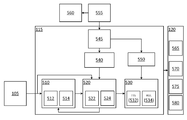•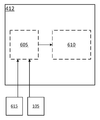•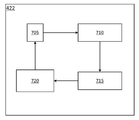•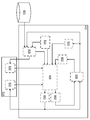•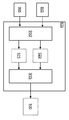•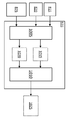•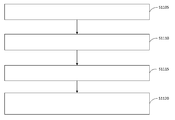•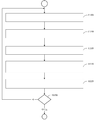•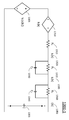•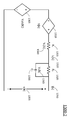•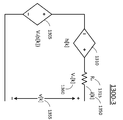•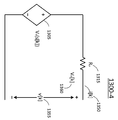•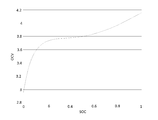•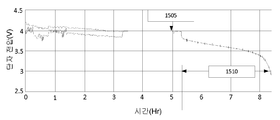•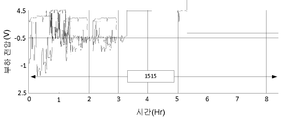•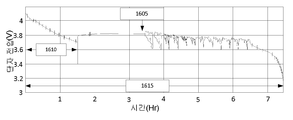•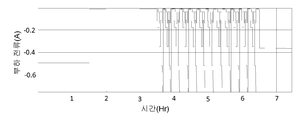•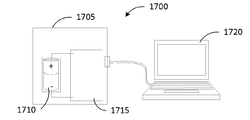•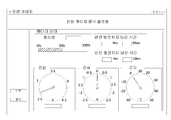•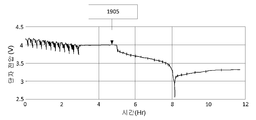•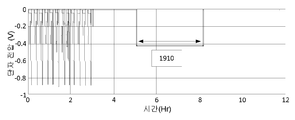•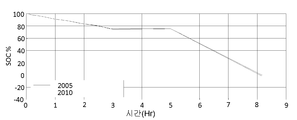••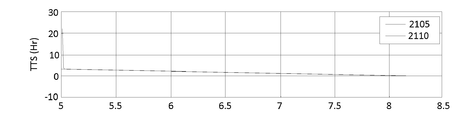•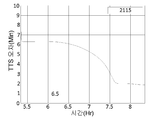•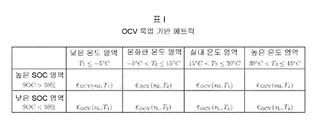•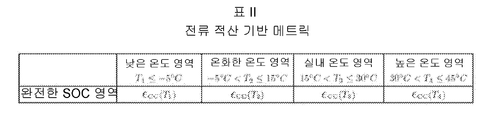•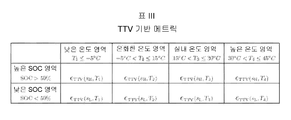## Classifications

• GPHYSICS
• G06COMPUTING; CALCULATING; COUNTING
• G06FELECTRIC DIGITAL DATA PROCESSING
• G06F30/30Circuit design
• G06F30/36Circuit design at the analogue level
• G06F30/367Design verification, e.g. using simulation, simulation program with integrated circuit emphasis [SPICE], direct methods or relaxation methods
• GPHYSICS
• G01MEASURING; TESTING
• G01RMEASURING ELECTRIC VARIABLES; MEASURING MAGNETIC VARIABLES
• G01R31/00Arrangements for testing electric properties; Arrangements for locating electric faults; Arrangements for electrical testing characterised by what is being tested not provided for elsewhere
• G01R31/36Arrangements for testing, measuring or monitoring the electrical condition of accumulators or electric batteries, e.g. capacity or state of charge [SoC]
• G01R31/367Software therefor, e.g. for battery testing using modelling or look-up tables
• GPHYSICS
• G01MEASURING; TESTING
• G01RMEASURING ELECTRIC VARIABLES; MEASURING MAGNETIC VARIABLES
• G01R31/00Arrangements for testing electric properties; Arrangements for locating electric faults; Arrangements for electrical testing characterised by what is being tested not provided for elsewhere
• G01R31/36Arrangements for testing, measuring or monitoring the electrical condition of accumulators or electric batteries, e.g. capacity or state of charge [SoC]
• G01R31/382Arrangements for monitoring battery or accumulator variables, e.g. SoC
• G01R31/3835Arrangements for monitoring battery or accumulator variables, e.g. SoC involving only voltage measurements
• GPHYSICS
• G01MEASURING; TESTING
• G01RMEASURING ELECTRIC VARIABLES; MEASURING MAGNETIC VARIABLES
• G01R31/00Arrangements for testing electric properties; Arrangements for locating electric faults; Arrangements for electrical testing characterised by what is being tested not provided for elsewhere
• G01R31/36Arrangements for testing, measuring or monitoring the electrical condition of accumulators or electric batteries, e.g. capacity or state of charge [SoC]
• G01R31/382Arrangements for monitoring battery or accumulator variables, e.g. SoC
• G01R31/3842Arrangements for monitoring battery or accumulator variables, e.g. SoC combining voltage and current measurements

## Abstract

The present method includes the steps of: calculating a first state of charge (SOC) of a battery at a first hour; receiving a voltage value representing the voltage measured in both ends of the battery at a second hour; calculating a filter gain in the second hour; and calculating a second estimated charging amount of the battery at the second hour based on a first estimated charging amount, the voltage value, and the filter gain. Another method includes the steps of: storing a library of equivalent circuit models representing a battery in a memory; determining the operation mode of the battery based on the load relevant to the battery; selecting one of the equivalent circuit models based on the determined operation mode; and calculating the charging amount of the battery by using the selected equivalent circuit model.

## Description

{BATTERY STATE OF CHARGE TRACKING, EQUIVALENT CIRCUIT SELECTION AND BENCHMARKING}

This application claims benefit of U.S. Provisional Patent Application No. 61 / 768,472 entitled " Methods and Apparatus Related to Battery Charging and Gauge Evaluation, " filed February 24, The entire contents of which are incorporated herein by reference.

Technical field

The present invention relates to the calculation of the battery charge amount.

Electrochemical storage plays an important role in future energy strategies. Indeed, batteries are a viable energy storage technology for today and in the near future. Devices powered by batteries, such as portable electronic equipment, mobile consumer electronics, aviation equipment, for example, are becoming more widespread. For example, accurate estimation of the charged amount of the battery may be difficult using previously known systems and methods. Thus, there is a need for a system, method, and apparatus for solving the shortcomings of current technology and providing other new and innovative features.

One embodiment includes a method. The method includes calculating a first estimated charged amount (SOC) of the battery at a first time, receiving a voltage value representing a measured voltage across the battery at a second time, calculating a filter gain at a second time, And calculating a second estimated charge amount of the battery at a second time based on the first estimated charge amount, the voltage value, and the filter gain.

Other embodiments include systems. The system includes a battery fuel gauge module configured to calculate an estimated charge amount (SOC) of the battery using a battery and a reduced order filter, wherein the reduced order filter is based on the previously calculated charge amount estimate And is a single state filter configured to recursively calculate an estimated charge amount.

Yet another embodiment includes a computer-readable medium comprising a code segment. When executed by a processor, the code segment is configured to cause a processor to perform a single state (SOC) configured to recalculate an estimated charge amount (SOC) of the battery, store the estimated charge amount in a buffer, and recalculate an updated estimated charge amount based on the estimated charge amount And uses the reduced order filter as a filter to calculate the updated estimated charge amount of the battery.

Yet another embodiment includes a method. The method includes storing a library of equivalent circuit models representing a battery in a memory, determining an operating mode of the battery based on a load associated with the battery, selecting one of the equivalent circuit models based on the determined operating mode And calculating a charge amount (SOC) of the battery using the selected equivalent circuit model.

Yet another embodiment includes a system. The system includes a data storage configured to store a library of equivalent circuit models representing a battery, a model selection module configured to select an equivalent circuit model based on the operating mode of the battery, ) Of the filter module.

Yet another embodiment includes a computer-readable medium comprising a code segment. When executed by a processor, the code segment causes the processor to select an equivalent circuit model from a library of equivalent circuit models representing the battery, based on the operating mode of the battery, .

BRIEF DESCRIPTION OF THE DRAWINGS Exemplary embodiments will be more fully understood from the following detailed description and the accompanying drawings, taken in conjunction with the accompanying drawings, in which like elements are denoted by the same reference numerals, and are for purposes of illustration only and thus are not limitations of the exemplary embodiments .
Figures 1 and 2 show a block diagram of a battery management system (BMS) in accordance with at least one exemplary embodiment.
3 shows a block diagram of signal flow for selecting a battery equivalent model in accordance with at least one exemplary embodiment.
4 shows a blow diagram of a signal flow for computing a battery charge amount (SOC) according to at least one exemplary embodiment.
5 shows a block diagram of a battery fuel gauge (BFG) system in accordance with at least one exemplary embodiment.
6 shows a block diagram of signal flow for a parameter module of a BFG system according to at least one exemplary embodiment.
7 shows a block diagram of signal flow for a SOC module of a BFG system in accordance with at least one exemplary embodiment.
8 shows a block diagram of an SOC module in accordance with at least one exemplary embodiment.
9 shows a block diagram of a full least squares (TLS) module of a SOC module according to at least one exemplary embodiment.
10 shows a block diagram of a recursive least squares (RLS) module of an SOC module according to at least one exemplary embodiment.
Figures 11 and 12 show a sequence flow diagram of a method according to at least one exemplary embodiment.
Figures 13A-13D show schematic diagrams of a battery equivalent model according to at least one exemplary embodiment.
14 shows the OCV-SOC characteristic curve of the portable Li-ion battery cell.
15A and 15B are graphs showing load profiles.
16A and 16B are graphs showing the simulated load profile.
Figure 17 illustrates an exemplary system implementation.
18 is a diagram illustrating a user interface that may be used with a system implementation.
Figures 19a and 19b include a graph illustrating an exemplary discharge voltage / current profile.
20A and 20B are graphs showing an exemplary current integration evaluation method.
21A and 21B are views showing a method of evaluating the shutdown time (TTS).
Figures 22A, 22B, and 22C are tables showing residual gauge readings.
These drawings are intended to illustrate the approximate nature of the methods and / or structures used in the specific illustrative embodiments and to supplement the description provided below. These drawings, however, are not to scale and do not necessarily reflect the exact structure or performance characteristics of any given embodiment, and are to be construed as defining or limiting a range of values or attributes included by the exemplary embodiment Can not be done. For example, the relative thickness and location of structural elements may be reduced or exaggerated for clarity.

The illustrative embodiments may include various modifications and alternative forms, but these embodiments are shown by way of example in the drawings and will be described in detail herein. It should be understood, however, that the intention is not to limit the exemplary embodiment to the specific forms disclosed, but on the contrary, the illustrative embodiments include all modifications, equivalents, and alternatives falling within the scope of the claims. Like reference numerals refer to like elements throughout the specification and drawings.

Accurate estimation of battery conditions, such as state of charge (SOC), state of health (SOH), and remaining useful life (RUL), is a reliable, It is important to use. Estimating these quantities is known as battery fuel gauging (BFG). Unlike the hydrocarbon fuels of many cars of today, the storage capacity of a battery is not constant. Generally, battery capacity varies with battery age, usage pattern, and temperature, thereby making BFG a challenging adaptive estimation problem and modeling battery characteristics over temperature, SOC variations, Requires line parameter identification.

Figures 1 and 2 illustrate a block diagram of a system 100 in accordance with at least one exemplary embodiment. 1, the system 100 includes a battery 105, a battery management system (BMS) 110, a display 120, an unlimited power source 125 (e.g., a wall outlet ), A car charging station, etc.), and a switch 130.

The BMS 110 may be configured to manage usage and / or status of the battery 105. For example, the BMS 110 may be configured to charge the battery 105 by using the switch 130 to connect or disconnect the unlimited power source 125 to or from the battery 105. For example, the BMS 110 may be configured to connect or disconnect a load (not shown) to the battery 105. For example, the BFG 115 may be configured to calculate the state of charge (SOC) and / or state of health (SOH) of the battery 105. SOC and / or SOH may be displayed on the display 120 (e.g., as a percentage, time remaining, etc.).

2, the BMS 110 includes at least analogue digital converters (ADCs) 205 and 220, filters 210 and 225, a digital amplifier 215, and a battery level gauge (BFG) . The BFG 115 includes a memory 230, a processor 235, and a controller 240. At least one of the ADCs 205 and 220, the filters 210 and 225, the digital amplifier 215 and the BFG 115 may be implemented as an application specific integrated circuit (ASIC), a digital signal processor (DSP) field programmable gate arrays, processors, and the like. Alternatively, the BMS 110 may be an ASIC, DSP, FPGA, processor, etc., including the functional blocks shown. Alternatively, the system 100 may be implemented as software stored in memory and executed, for example, by a processor.

The BMS 110 uses a combination of digital converters (ADCs) 205 and 220, filters 210 and 225 and digital amplifier 215 to convert analog measurements (e.g., I b and V b ) (E.g., for use by BFG 115 to compute SOC and / or SOH). For example, the digital amplifier 215 may generate an analog signal based on the voltage drop V b across the battery 105 (e.g., the difference between the voltage values between the positive and negative terminals) ) Differential amplifier, and this analog signal is then converted to a filtered digital value using the ADC 220 and filter 225.

System 100 may be any system or subsystem of an electronic device that uses a battery to provide power. In some implementations, the electronic device may be or be, for example, a laptop-type device having an existing laptop-type form factor. In some implementations, the electronic device may be, for example, a wired device and / or a wireless device (e.g., a Wi-Fi enabled device), a computing entity (e.g., a personal computing device), a server device A cellular phone, an audio device, a motor control device, a power supply (e.g., an off-line power supply), a personal digital assistant (PDA), a tablet device, an e-reader, . In some implementations, the electronic device may include a display device (e.g., a liquid crystal display (LCD) monitor, to display information to a user), a keyboard, a pointing device , A device through which a user can provide input to a computer).

FIG. 3 shows a block diagram 300 of signal flow for selecting a battery equivalent model in accordance with at least one exemplary embodiment. 3, model selection block 310 receives input 320 (e.g., a voltage and / or current signal from a battery and / or a load) and inputs 320 (or some variations thereof ) To select an equivalent model representing the battery (or corresponding to the battery) from the equivalent model library 305. [ The equivalent model is then used to calculate the charge quantity (SOC) by the charge quantity calculation block 315. [ The equivalent model library 305 may include at least one equivalent model representing a battery. Each equivalent model may be based on the operating mode of the battery (or equivalent battery). The mode of operation may be based on the load associated with the battery. For example, the operating mode may be based on a voltage drop across the load. For example, the mode of operation may be based on whether the voltage drop across the load is relatively high or low, relatively static or dynamic, and / or a combination thereof.

The equivalent model (see Figures 13a-13d below) may include a resistor, a voltage (e.g., a voltage drop or voltage source), a resistive-current (RC) circuit, an impedance circuit, and / or the like. Therefore, a mathematical (e.g., formal) equivalent to an equivalent model of a battery can be developed. The equivalent model library 305 may store mathematical equivalents in relation to the operating mode of the battery. Mathematical equivalents may be used to calculate the SOC by the charge computation block 315. [ For example, a mathematical equivalent may be used to determine variables as inputs to a formula used to calculate an SOC (or an estimate of SOC). Therefore, the BFG system can improve the computation efficiency and reduce the processing time by selecting the equivalent model based on the operation mode. A more detailed description is provided below with respect to Figures 5-12.

FIG. 4 shows a block diagram 400 of a signal flow for computing a battery charge amount (SOC) in accordance with at least one exemplary embodiment. 4, the block diagram 400 includes an extended Kalman filter (EKF) block 405, a SOC block 410 and 420, a filter gain parameter block 415 and 425, And a buffer 430. The EKF block 505 computes the SOC 420 based on the previously computed SOC 410 and the filter gain parameter 415 and computes the filter gain parameters 425 (e.g., the read and / or the computed SOC variance variance, measured voltage, calculated capacity, variables associated with equivalent circuits, and / or the like). Accordingly, the buffer 430 may be configured to store the previously calculated SOC and filter gain parameters in, for example, a processing loop. That is, the current (or next) SOC may be computed based on at least one SOC computed earlier. In other words, The SOC calculated at the first time can be used to calculate the SOC at the second time (next).

In an exemplary implementation, at least two SOC 410 and / or a set of filter gain parameters 415 may be used. Thus, the vector of at least two SOCs, the array of at least two SOCs, the average of at least two SOCs, and at least two intermediate values, and the corresponding filter gain parameter, SOC at the second time) and to determine / calculate the corresponding filter gain parameter 425. [ Accordingly, the buffer 430 may be configured to store the plurality of previously computed SOCs 410 and the previously computed / determined filter gain parameters 415. [ Therefore, the BFG system can improve the computation efficiency and reduce the processing time by using the previously calculated SOC. A more detailed description is provided below with respect to Figures 5-12.

FIG. 5 shows a block diagram of a battery remaining gauge (BFG) 115 system in accordance with at least one exemplary embodiment. 5, the BFG 115 includes an estimation module 510, a tracking module 520, a prediction module 530, an open circuit voltage-state of charge (OCV- (SOC) characterization module 540, an offline parameter estimation module 545, and a battery life characteristics analysis module 550. The system also includes an offline data collection module 555 and a battery modeling module 560.

The offline data acquisition module 555 may be configured to measure battery characteristics in a relatively controlled test environment. For example, an open circuit voltage (OCV) measurement and an SOC measurement may be collected for a battery 105 (or an equivalent battery) in a test laboratory environment. For example, the battery 105 (or equivalent battery) may be initialized to a fully charged (e.g., almost fully charged, substantially fully charged), rested state. OCV and SOC measurements can be made. OCV and SOC measurements are then made at intervals (e.g., regular intervals, periodic intervals, irregular intervals, predetermined intervals) until the battery 105 (or equivalent battery) is completely (or substantially) 105 (or an equivalent battery) may be gradually discharged. The OCV and SOC measurement values are used to determine and calculate battery parameters (e.g., OCV parameters K i ∈ {K 0 ; K 1 ; K 2 ; K 3 ; K 4 ; K 5 ; K 6 ; K 7 } Or estimating the amount of water.

Data from the off-line data collection module 555 may be used in the battery modeling module 560 to determine, for example, an equivalent model for the battery 105 (or equivalent battery) and / or a mathematical equivalent to such an equivalent model . The data from the off-line data collection module 555 is stored in the off-line parameter estimation module 545 to determine and / or calculate the parameters associated with the battery 105 (e.g., the values for the components associated with the aforementioned equivalent models) Can be used. The data from the off-line data collection module 555 includes OCV and SOC battery parameters (e.g., OCV parameters K i ∈ {K 0 ; K 1 ; K 2 ; K 3 ; K 4 ; K 5 ; K 6 ; SOC characteristic analysis module 540 to determine and / or calculate the output signal (e.g., K 7 ). Data from the offline parameter estimation module 545 may be used in the battery life characteristics analysis module 550. For example, data from the off-line parameter estimation module 545 may be used to calculate an initial SOH characteristic (e.g., maximum SOC) by the battery life characterization module 550. [

The display 120 is shown having a SOC display 565, an SOH display 570, a time to shutdown (TTS) display 575, and a remaining useful life display 580. Each display may be, for example, a meter showing a percentage. The values for each display may be calculated or determined by the BFG 115. For example, the TTS may be displayed as a time value (e.g., time and / or minute) computed by the TTS module 532.

The estimation module 510 includes a parameter module 512 and a capacity module 514. The estimation module 510 may be configured to calculate and / or determine specific values (e.g., parameters and capacity values) for the battery 105 (or equivalent battery). In a stable environment (e.g., a test lab), parameter and capacity values can be fixed (i.e., unchanged). However, in a real environment, the parameter and capacity values can be dynamic or variable. For example, a complete SOC tracking solution generally includes (1) an estimate of OCV parameters that form part of the state space model through offline OCV characterization. OCV-SOC characterization is stable against temperature changes and battery aging. Once estimated, these parameters form part of the state-space model with known parameters. A complete SOC tracking solution generally includes (2) estimating dynamic electrical equivalent circuit parameters. These parameters have been observed to vary with temperature, SOC and battery life, and for this reason should be estimated adaptively during operation of the BFG. A complete SOC tracking solution generally includes (3) estimating the battery capacity. Although the nominal capacity of a battery is specified by the manufacturer, the available battery capacity is known to vary due to errors in the manufacturing process, temperature variations, usage patterns, and aging. And a complete SOC tracking solution generally includes (4) model parameters - conditional SOC tracking. Once the model parameters are known, SOC tracking becomes a non-linear filtering problem. However, it is observed that the resulting state-space model includes correlated processes and measurement noise processes. Properly resolving the effects of these correlations will yield better SOC tracking accuracy. Thus, in an exemplary implementation, the tracking module 520 may feed back data to the estimation module 510 to calculate the parameters and capacity.

In addition, common methods for estimating battery capacity assume that the rested battery voltage is indicative of the battery's true open circuit voltage (OCV), ignoring the hysteresis effect. However, according to an exemplary embodiment, the estimation module 510 models the hysteresis as an error in the OCV of the battery 105 and employs a combination of real time, linear parameter estimation, and SOC tracking techniques to compensate for the error in the OCV do.

The tracking module 520 includes a SOC module 522 and an SOH module 524. The SOC represents the amount of "fuel" in the battery 105. As previously described, SOC is the available capacity expressed as a percentage of some criterion (e.g., rated capacity or current capacity). According to an exemplary embodiment, the SOC module 522 compensates for errors in the OCV (in combination with parameter estimation), which is described in more detail below by calculating the SOC using tracking. The SOH represents the state of the battery compared to a new or ideal battery. The SOH may be based on charge acceptance, internal resistance, voltage, self-discharge and / or the like.

The prediction module 530 includes a TTS module 532 and a RUL module 534. TTS module 532 and RUL module 534 may be configured to calculate TTS and RUL based on the SOC.

FIG. 6 shows a block diagram of signal flow for a parameter module 412 of a BFG 115 in accordance with at least one exemplary embodiment. The BFG system can improve computation efficiency and reduce processing time by selecting an equivalent model based on the operation mode. As shown in FIG. 6, the parameter module 412 includes an operation mode module 605 and a model selection module 610. The operating mode module 605 may be configured to determine an operating mode (of the battery) based on at least one input from the battery 105 and / or at least one input from the load 615. [ The at least one input may be based on at least one of a current and a voltage associated with at least one of the battery 105 and the load 615. For example, the operating mode may be based on a voltage drop across the load 615. [ For example, the mode of operation may be based on whether the voltage drop across the load is relatively high or low, relatively static or dynamic, and / or a combination thereof. The model selection module 610 may select an equivalent model (or a mathematical equivalent thereof) based on the determined operation mode. For example, the model selection module 610 may generate a query term that is used to search for the equivalent model library 305.

In some implementations, several modes of operation may be defined or characterized. In an exemplary embodiment, four modes of operation associated with a battery and a system using such a battery are described below.

In the first mode of operation, the battery 105 may be connected to a heavy and varying load. In other words, the load 615 may utilize a relatively high voltage with dynamic or variable current consumption (or a high voltage load that consumes variable current). For example, in a mobile phone, the first mode of operation may include a usage environment in which the mobile phone use includes long time video playback, multimedia and gaming applications, and the like. The equivalent circuit shown in FIG. 13A below may represent a battery connected under a variable load.

In the second mode of operation, the battery 105 may be connected to a dynamic load and / or a variable voltage load. In other words, the load 615 may utilize a dynamic voltage or a variable voltage. For example, in a mobile phone, the second mode of operation may include a usage environment in which mobile phone use includes regular use for phone calls, web browsing and / or video clip playback. The equivalent circuit shown in Fig. 13B below may represent a battery connected to a dynamic load.

In the third mode of operation, the battery 105 may be connected to a load that consumes a constant current. In other words, the load 615 is a constant (or substantially constant) Current can be consumed. Alternatively, the battery 105 may be charged using a constant current. For example, the battery 105 may be disconnected from the load 615 during a charging cycle (e.g., the unlimited power supply 125 may be connected to the battery 105 using the switch 130, Can be charged). The equivalent circuit shown in Fig. 13C below may represent a battery connected to a constant current.

In the fourth mode of operation, the battery 105 may be connected to a relatively low voltage load. Alternatively, the battery 105 may be in a periodic resting state following light loading followed by charging, then rest, minimal load or no load. In other words, the load 615 may seldom use the minimum voltage. For example, in a mobile phone, the fourth mode of operation includes a usage environment in which cellular phone use includes regular pings of the transmission tower with rare phone calls after a full charge (or substantially full charge). The equivalent circuit shown in FIG. 13D below may refer to a battery connected to a low voltage load.

FIG. 7 shows a block diagram of signal flow to the SOC module 422 of the BFG 115 in accordance with at least one exemplary embodiment. 7, the SOC module 422 includes a buffer block 705, a model estimation block 710, an SOC tracking block 715, and a voltage drop prediction module or block 720.

In the exemplary embodiment, hysteresis is modeled as an error in the OCV of the battery 105. The voltage drop v D [k] can represent the voltage across the battery model internal components R 0 , R 1 , R 2, and x h [k] (see FIG. 13a). The term x h [k] can be used to account for the error in the expected SOC. That is, x h [k] may be an "instantaneous hysteresis" which can be corrected to zero by adjusting the computed or estimated SOC. If the computed or estimated SOC is equal to SOC, the computed or estimated x h [k] should be equal to zero. In other words, the non-zero calculated or estimated x h [k] represents the error in the computed or estimated SOC. The voltage drop model parameter vector ( b ) contains an element corresponding to the computed or estimated x h [k].

7, the computed or estimated current SOC from the SOC tracking block 715 is used in the voltage drop prediction block 720 to compute the voltage drop v D [k]. At least one past voltage drop v D [k] is stored in the buffer 705 and used to estimate the parameter vector b . The non-zero value of the corresponding computed or estimated x h [k] in the parameter vector b indicates the presence of instantaneous hysteresis. This means the SOC estimation error. The SOC tracking algorithm of the SOC tracking block 715 is configured to modify the SOC whenever the computed or estimated x h [k] is non-zero. Additional details regarding voltage drop v D [k], hysteresis, estimated x h [k], voltage drop model parameter vector ( b ) and SOC tracking are described below (mathematically). As described above, the BFG system can accurately estimate the SOC, improve the calculation efficiency, and reduce the processing time by using the calculated SOC and the SOC error.

FIG. 8 shows a block diagram of a SOC module 422 in accordance with at least one exemplary embodiment. As shown in FIG. 8, the SOC module 422 includes an extended Kalman filter (EKF) block 805. The EKF block may be configured to calculate SOC 845 and SOC error 840. The EKF block 805 computes the SOC 845 using the equation (1) as the estimated SOC and computes the SOC error 840 as the estimated SOC error (or variance) using the equation (2) . K represents an instantaneous iteration, k + 1 | k represents the last, previous or previous iteration, and k + 1 | k + 1 represents the current, update, next, or subsequent iteration .

here,

Is the estimated SOC for the current or update iteration;

Is the estimated SOC for the last or expected repeat;

Is the filter gain for the last or anticipated iteration;

Is the load voltage for the last or expected repeat.

here,

Is the SOC estimation error or variance for the current or update iteration;

Is the filter gain for the last or anticipated iteration;

Is a linearized observation coefficient;

Is the SOC estimation error or variance for the last or expected repeat;

Is a voltage drop noise with zero mean and correlation at initialization.

The SOC module 422 includes an OCV parameter block 810. The OCV parameter block 810 may be configured to store and / or receive the OCV parameters {K i } from the OCV-SOC characteristic analysis module 540. The OCV parameters {K i } are constant in that they are measured off-line and the change in lifetime of the battery 105 is negligible (or nonexistent). The OCV parameter is used to calculate the OCV with respect to SOC according to equation (3).

here,

Is the SOC;

Is an open circuit voltage (OCV).

The SOC module 422 includes a voltage drop model block 825. The voltage drop model block 825 can be configured to calculate the voltage drop across the load using the voltage drop model (previously described) according to equation (4) or (5).

here,

Is the measured voltage;

Is an open circuit voltage (OCV);

Is a voltage drop model;

Is the voltage drop model parameter vector;

Is an estimated voltage drop model;

Is the estimated voltage drop model parameter vector;

Is the voltage drop observation noise.

The voltage drop model can be varied based on the selected equivalent circuit model as described above. The selected equivalent circuit model and / or voltage drop model may be read from the data storage 855. For example, the data storage 855 may include an equivalent model library 305.

The EKF (module or) block 805 may be configured to use the equation (1) to calculate the SOC 845 as the estimated SOC and store the resulting SOC 845 in the buffer 850. EKF block 805 may be configured to calculate SOC error 840 as an estimated SOC error (or variance) using equation (2) and store the resulting SOC error 840 in buffer 850 . The stored SOC and SOC errors can be read as SOC 815 and SOC error 820. Accordingly, the EKF block may determine that the subsequent (update, next, and / or temporally subsequent) SOC 845 and SOC error 840 operations are performed by at least one previous (current, last or temporal) SOC 815 (E. G., In a loop) the SOC 845 and the SOC error 840 so that the SOC 845 and the SOC error 820 may be based on an SOC error 820 operation.

8, a Recursive least squared (RLS) block 830 and a Total Least Squared (TLS) block 835 may generate an input to the EKF block 805 have. The RLS block may generate an initial estimated voltage drop model parameter vector (which may include at least one voltage drop model parameter) and the TLS block 835 may generate an initial estimated capacity. This initial estimated voltage drop model parameter vector and initial estimated capacity can be generated for each loop. In an exemplary implementation, as the number of iterations k increases, the initial estimated voltage drop model parameter vector and the change in the initial estimated capacity may be negligible.

9 is a block diagram of a full least squares (TLS) block 835 of the SOC module 422 in accordance with at least one exemplary implementation. As shown in FIG. 9, the TLS block 635 includes a buffer 910 and a TLS operation module 915. Buffer 910 is configured to receive, store, and output SOC data, e.g., SOC change (or change) data 920, to be used by TLS operation module 915. The buffer 910 is further configured to receive, store, and output current variation (or change) data 925 to be used by the TLS computation module 915. Buffer 910 may receive current data 905 as coulomb counting data based on the measured current associated with battery 105, for example.

The TLS operation module 915 can be configured to calculate the capacity 930 of the battery 105 based on the SOC change amount 920 and the current change amount 925. [ For example, the TLS operation module 915 may calculate the capacity 930 using Equation (6). The deviation of Equation (6) will be described in more detail below.

here,

Is the estimated capacity;

Is the covariance of the augmented observation matrix;

Is a 2 * 2 diagonal matrix with nonnegative eigenvalues.

10 is a block diagram of a recursive least squares (RLS) block 830 of the SOC module 422 in accordance with at least one exemplary embodiment. As shown in FIG. 10, the RLS block 635 includes a buffer 1005 and an RLC operation module 1010. Buffer 1005 is configured to receive and store SOC 815, SOC error 820 and voltage drop data (e.g., Z v [k] or OCV) as an output from voltage drop model block 825. The buffer 1005 is configured to output a voltage drop 1015 and a current and capacitance (I & C) matrix 1020.

The RLC computation module 1010 may be configured to compute an initialization parameter 1025 based on the voltage drop 1015 and the I & C matrix 1020. For example, the RLC computation module 1010 may compute an initialization parameter 1025 using Equation (7). The deviation of the equation (7) will be described in more detail below.

Where a i is the current attenuation coefficient in the R 1 C 1 circuit,

β i is the current attenuation coefficient in the R 2 C 2 circuit,

Is the estimated resistance value of R 1 ;

Is the estimated resistance value of R 2 ;

Is the estimated hysteresis voltage of the battery;

Is instantaneous hysteresis.

As described above with respect to FIG. 7 above, the estimated hysteresis voltage of the battery will be zero. Thus, in an exemplary implementation, b (6) in Equation (7) can be computed using SOC tracking block 715 to eliminate (or substantially eliminate) the error due to hysteresis using SOC tracking. Will be. Therefore, the hysteresis can be considered, so the SOC estimation is more accurate.

In FIGS. 8-10, the length of the buffer 1005 may be L b for parameter estimation, and the length of buffer 905 may be L c for capacity estimation. The EKF block 805 is repeated every k, and the RLS 830 is repeated every k that is an integer multiple of L b , and the TLS 835 is repeated every k times an integer multiple of L c , where k is a time index . The BFG shall be capable of measuring all required model parameters, battery capacity required for SOC tracking, and voltage and current measurement error standard deviation (resulting from calibration of the measuring instrument circuit), excluding OCV parameters

). The RLS block does not require any external initial conditions - just set the lambda = 1 to provide a robust LS estimate as an initial value, And Lt; / RTI &gt; And Is the batch number. The mathematical proof of the EKF block 805 is described below.

11 and 12 are flowcharts of a method according to at least one exemplary embodiment. The steps described in connection with Figures 11 and 12 may be stored in a memory (e.g., memory 230) associated with a device (e.g., BMS 110 shown in Figures 1 and 2) and associated with at least one (E. G., Processor 235) of the computer system 100. The computer system 100 may be implemented with software code executed by a processor (e. However, alternative implementations such as systems implemented as dedicated processors are also contemplated. The steps described below are described, for example, as being executed by a processor, but these steps are not necessarily executed by the same processor. In other words, at least one processor may perform the steps described below with respect to Figures 11 and 12.

11 is a flowchart of a method for selecting an equivalent model representing a battery for use in computing an estimated SOC. As shown in Fig. 11, in step S1105, a library of an equivalent circuit model representing a battery is stored in a memory. For example, data associated with the battery 105 (or equivalent battery) may be collected using the offline data collection module 555. Using data and common circuit tools, at least one equivalent circuit representing the battery can be created. Such an equivalent circuit may comprise any combination of at least one equivalent voltage, resistance, capacitance, and / or impedance equivalent. For example, reference can be made to Figures 13A-13D below. Mathematical equivalents for each equivalent circuit can also be generated. Equivalent circuits and / or mathematical equivalents may be stored, for example, in the equivalent model library 305.

In step S1110, the operating mode of the battery is determined based on the load associated with the battery. For example, each equivalent model may be based on the operating mode of the battery (or equivalent battery). The mode of operation may be based on the load associated with the battery. For example, the operating mode may be based on a voltage drop across the load. For example, the mode of operation may be based on whether the voltage drop across the load is relatively high or low, relatively constant or dynamic, and / or a combination thereof. Therefore, the operating mode can be determined based on the current and / or voltage associated with the battery and / or the load associated with the battery.

In step S1115, one of the equivalent circuit models for the determined mode is selected based on the determined mode. For example, the equivalent model library 305 may be searched based on the determined operation mode. For example, equivalent circuitry and / or mathematical equivalents representing the battery may be stored in the equivalent model library 305 consistent with an operating mode identifier (e.g., a unique name or unique identification number). Thus, the determination of the operating mode may include determining an operating mode identifier that is then used to search for the equivalent model library 305. [ The selection of an equivalent circuit may include selecting an equivalent circuit or mathematical equivalent that is returned by the search of the equivalent model library 305.

In step S1120, the charge amount SOC of the battery or the estimated SOC is calculated using the selected equivalent circuit model. For example, as described above, the operation of the SOC may be based on the voltage drop model parameter vector (b). The voltage drop model parameter vector may have parameters based on the equivalent circuit of the battery (see equation (7) above). Thus, the determined voltage drop model parameter vector can be somewhat complex based on an equivalent circuit. For example, as described below, an equivalent circuit may not include an RC circuit element because the capacitor is charged to bypass the resistor. Thus, b (3) may be the only remaining voltage drop model parameter vector element. In this way, the calculation of SOC or estimated SOC is simplified. Further, the voltage v [k] across the terminal of the battery 105 that can be used to calculate the SOC or the estimated SOC may be based on the equivalent circuit model. v [k], SOC, and the equivalent circuit model will be described in more detail below.

12 is a flowchart of a method for calculating an estimated SOC using a cyclic filter. As shown in Fig. 12, in step S1205, the stored estimated charge amount SOC of the battery is read from the buffer. For example, the buffer 850 may have stored at least one SOC error, and the SOC computed in a previous iteration of the steps described in connection with such a flowchart. At least one of the stored SOC values may be read from the buffer 850.

In step S1210, the measured voltage across the battery is read. For example, a voltage (e.g., v [k] shown in Figures 13A-13D below) may be read or determined, for example, using digital amplifier 215. [ In one exemplary implementation, this voltage is stored in a buffer. Thus, different iterations can use different voltage measurements. In other words, a voltage measurement prior to (in time) may be used in the current iteration, or v [k + 1] may be used in iteration (k + 2).

In step S1215, the filter gain is calculated. For example, a filter gain (e.g., G [k + 1]) for EKF block 805 is computed, as described briefly above and described in more detail below. The filter gain may be based on at least one capacity value computed using a weighted least squares algorithm. For example, the filter gain may be based on at least one capacity value computed using at least one of a weighted recursive least squares (RLS) algorithm and a full least squares (TLS) algorithm. The filter gain may be based on the capacity value computed using the weighted RLS algorithm based on the SOC tracking error covariance and current measurement error standard deviation. The filter gain may be based on the estimated SOC variance. The filter gain may be based on a capacity value computed using the TLS algorithm based on a recursive update of the covariance matrix. The filter gain may be based on a capacitance value computed using an open circuit voltage (OCV) lookup. (E.g., mathematically) for each of the SOC tracking error covariance, current measurement error standard deviation, SOC variance, covariance matrix and OCV.

In step S1220, the estimated SOC of the battery is calculated based on the stored SOC of the battery, the voltage across the battery, and the filter gain. For example, the estimated SOC may be equal to the stored estimated SOC plus digital voltage value multiplication filter gain. In step S1225, the estimated SOC computed is stored in a buffer (e.g., buffer 850). If additional computation of the estimated SOC is required and / or desired (S1230), the process returns to step 1205. [ For example, if the battery 105 is still in use, the SOC error exceeds the required value and additional iterations can reduce the error, additional testing is needed if the battery test is in progress and / or the like And / or may be desirable.

Figures 13A-13D show schematic diagrams of a battery equivalent model according to at least one exemplary embodiment. Reference will now be made to Figures 13a-13d, where necessary, to describe one or more exemplary implementations. 13A-13D, an equivalent model representing the batteries 1300-1, 1300-2, 1300-3, 1300-4 includes resistors 1315, 1325, 1340, capacitors 1330, 1345, And may include any combination of equivalent voltage sources 1305 and 1310. Voltage 1355 represents the voltage drop across the battery when loaded. Currents 1320, 1335, and 1350 represent currents flowing through (or into) the elements of the equivalent model. For example, current 1350 represents the current flowing into the load.

The resistor and the capacitor can form an RC circuit. For example, resistors 1325 and 1330 form an RC circuit. In some exemplary embodiments, the capacitors may be fully charged and short-circuited, causing the RC circuit to substantially disappear from the equivalent model. For example, the RC circuit formed by the resistor 1340 and the capacitor 1345 in the equivalent model representing the battery 1300-2 is not in the model because the capacitor 1345 is fully charged to form a short circuit to be. In some exemplary implementations, there is no hysteresis (or minimal hysteresis) associated with the battery (e.g., the battery is in a non-operating state or only a minimal load). Therefore, as shown in the equivalent model representing the battery 1300-4, the equivalent voltage source 1310 is not in the model, because there is no hysteresis.

The present disclosure continues by describing details of an exemplary embodiment. Such details may include the evolution of at least one of the above equations (e.g., mathematical proof or simplification). The mathematical formula may be repeated for clarity, but such mathematical formulas will hold the mathematical formula number indicated within square brackets ([]). We start with real-time model identification, which can refer to the following notation.

Observation model

In a matrix (κ) Continuous observation of

Observation model parameters

LS estimation of model parameters

RLS estimation of model parameters

Current through battery

OCV parameters:

The length of the arrangement for parameter estimation

Current measurement error

Voltage measurement error

The covariance matrix of the LS estimator

Battery internal series resistance

Battery internal resistance in circuit

Battery internal resistance in circuit

Voltage across battery

Voltage drop

The k-th arrangement of consecutively observed voltage drops in vector form

Z-transform of voltage drop

Current through

Current through

Charge amount (SOC)

Estimate of

Measured current through battery

Current measured across the battery

Sampling time

LS fitting error

Percent estimation error of

Percent estimation error of

Voltage Drop Observation Noise Covariance

The elements of the SOC tracking algorithm may include the following:

a. Estimation of OCV parameters: OCV-SOC characterization is stable over time of battery temperature change and use period when normalized by age and age dependent battery capacity.

b. Estimation of Dynamic Electrical Equivalent Circuit Parameters: These parameters are observed to vary with the temperature of the battery, the SOC and the duration of use, and therefore must be estimated adaptively during operation of the BFG.

c. Estimation of battery capacity: Although the nominal capacity of a battery is specified by the manufacturer, it is known that the available battery capacity varies due to errors in the manufacturing process, temperature changes, load patterns, and the use period.

d. Model Parameter-Controlled SOC Tracking: Once the model parameters are known, SOC tracking becomes a non-linear filtering problem.

The exemplary embodiment enables real-time linear estimation of dynamically equivalent circuit parameters for a battery. Improvements to existing approaches for battery equivalent circuit modeling and parameter estimation are made by addressing the following issues in this illustrative embodiment:

a. Some models take into account resistance-only and are inadequate for dynamic loads.

b. These models employ a nonlinear approach for system identification.

c. Initial parameter estimates are needed for the model identification method.

d. It is assumed that a single dynamic equivalent model represents all battery operating modes.

In this embodiment, the four issues are addressed and summarized as follows:

a. An on - line linear approach for model parameter estimation without estimating the parameters of the exact physical representation of the battery equivalent circuit. The SOC tracking state-space model leverage the estimation of the modified parameters, which can be estimated linearly.

b. Applicable to a wide variety of batteries without requiring any initialization or calibration: Due to the adaptability of the exemplary state-space model, the proposed SOC tracking approach does not require any offline initialization of the model parameters. The least squares (LS) method provides the initialization (or re-initialization) of the parameters as needed, and the block recursion least squares (RLS) is employed to continuously track the model parameters. Further, it can be seen that the modified open circuit voltage (OCV) model is valid over different battery models, different temperatures, and different load conditions. This allows the exemplary BFG to be applied in a plug-and-play manner over a wide range of batteries without requiring any additional information about the battery.

c. Possibility of integrity SOC tracking through different modes of battery. Four different battery equivalent models can be identified to reflect very low load or no-run conditions, constant current or low frequency load, dynamic load and varying overload. Four (slightly) different dynamic equivalent models may also be identified to best suit these modes. This model can be used for integrity SOC tracking regardless of mode changes in battery operation.

d. Hysteresis Modeling Eliminating the Need for Hysteresis Modeling: In the exemplary embodiment, since hysteresis is a function of SOC? [0 1] and load current I? R, it is almost impossible to model hysteresis offline (perfectly) Recognize points. Hence, according to an exemplary embodiment, in a voltage drop model, hysteresis is modeled as the error of the OCV, and the on-line filtering approach attempts to continually reduce the difference by adjusting the SOC (with the correct value).

Real-time model identification includes real-time model parameter estimation using an equivalent circuit. 13A is an equivalent circuit of an exemplary battery (e.g., battery 105). When the battery is inactive, V 0 (s [k]) is the OCV of the battery. This OCV is uniquely dependent on the SOC of the battery (s [k] ∈ [0 1]). When the battery is activated, for example, when the current activity, the operation of the battery is the hysteresis component (h [k]), the series resistance (R 0), and each of the two parallel series connected RC circuit, that is, (R 1 , C 1 ) and (R 2 , C 2 ). The discrete time is represented using [k].

In Figure 13A, the measured current through the battery is:

Here, i [k] is the actual current through the battery, n i [k] is the current measurement noise, and this current measurement noise is estimated to be 0 on average and has a standard deviation sd of σ I. The measured voltages across the battery are:

It is assumed that v [k] is the actual voltage across the battery, n v [k] is the voltage measurement noise, and this voltage measurement noise has an average of zero and a standard deviation of σ V.

The voltage drop and h [k] of the battery across the internal components (R 0 , R 1 , R 2 ) are given by:

Here the current through resistors R 1 and R 2 can be expressed in the following form:

here,

Δ is the sampling interval.

By replacing i [k] with the measured current z i [k], the currents in equations (11) and (12) can be expressed as:

Now, equations (8) and (10) can be expressed in the z-domain as:

Next, equation (15) can be expressed in the z-domain as: &lt; RTI ID = 0.0 &gt;

As a result,

, And for Equation (16) as well,

.

Substituting Equations (19) and (20) into Equation (17):

.

If we re-arrange equation (21) and convert it back to the time domain:

here,

to be.

Now, equation (22) can be rewritten as: &lt; RTI ID = 0.0 &gt;

Here, the observation model a [k] T and the model parameter vector b are given by the following equations:

Here, the suffix "4" indicates the model corresponding to the model 4 among the four models shown in Figs. 13A-13B.

The noise in the voltage drop observation in equation (30) is expressed as: &lt; RTI ID = 0.0 &gt;

It has autocorrelation given by the following equation:

The hysteresis component is assumed to be a constant during the placement of the time interval of length L b , for example:

Now, four different "modes" of battery operation and an appropriate battery equivalence model corresponding to these modes can be described.

a. Mode 1 - Low load or non-operating state: If the battery is only in a low load state, then charged and then inactive, the hysteresis component will be negligible. As an example of this mode, there will be a cellular phone that, after being fully charged, pings for most of the time, except for the possibility of very few phone calls until the next charging. A single resistor (see Figure 13d) fits this mode well.

b. Mode 2 - Constant Current Operation: When the current through the battery is constant, the capacitor of the RC circuit is fully charged. Therefore, in terms of parameter estimation, the resulting circuit can be regarded as a single resistor and a hysteresis / bias component (see FIG. 13C). Constant current charging of the battery is a good example of this mode.

c. Mode 3 - Dynamic Load: When the battery is in this mode, there is a significant amount of significant load. For example, there are smartphones that are regularly used for phone calls, web browsing, video clips, and so on. The battery equivalents shown in FIG. 13B fit this scenario well.

d. Mode 4 - Overload and changing usage: For mobile phones, overload and changing usage include long-term video playback, multimedia and gaming applications. Figure 13a fits this scenario.

It should be noted that the different model complexities of the dynamically equivalent circuit can be represented by simply changing [k] T. The definition of [k] T for each model can be seen below. For each of the model complexities, the noise term n D [k]

And Lt; / RTI &gt;

here,

to be.

The following discussion relates to least squares estimation of time-invariant dynamic model parameters. The actual SOC at time k is expressed as:

An advanced SOC tracking algorithm is used to update the updated estimate of x S [k]

Can be obtained. Now, the voltage drop v D [k] in equation (10) can be expressed as:

here,

Represents the estimated open circuit voltage (OCV) of the battery which can be described as a function of the estimated SOC. The following OCV-SOC relationship can be used:

OCV parameter

Can be estimated offline through the procedure described in detail below. By considering the placement of Lb observations, equation (30) can be rewritten as: &lt; RTI ID = 0.0 &gt;

Here, κ is a batch number,

to be.

Then,

Has the following covariance:

here,

Is a pentadiagonal Toeplitz matrix, and for this matrix the diagonal, first and second hypertext entries are (See equation (32)). Now, the dynamic model parameter vector can be estimated from equation (42) through least squares (LS) optimization as:

The covariance matrix of the LS estimator is given by:

If a new measurement arrangement is obtained, the LS estimate can be recursively updated by repeating equations (50) to (51): &lt; EMI ID =

(·) T denotes a transposed matrix, (·) -1 denotes an inverse matrix,

Is known as an information matrix that can be initialized by multiplying an appropriate size unit matrix by an appropriate constant. When? = 0, The . There may be several ways to approximate. The following two approximations can be chosen for comparison:

a. The following approximations can be made:

b. The previous estimate is used to construct the current covariance matrix, for example using the following equation: &lt; RTI ID = 0.0 &gt;

The following discussion relates to the minimum mean square error (MMSE) estimation of time varying dynamic model parameters. It is assumed that the dynamic model parameters are random variables that go through the following Wiener process:

here,

Covariance Gt; 0 &lt; / RTI &gt; white Gaussian noise. Now, using equation (30) as the measurement model and using equation (53) as the process model, the Kalman filter provides an MMSE estimate of b. (See equation (42)). &Lt; / RTI &gt; In order to increase the accuracy in SOC tracking and parameter estimation, iterative schemes of SOC tracking / smoothing and Kalman filtering based parameter estimation over a sufficiently long observation window can be used.

The following discussion relates to the associated open circuit voltage (OCV) parameter estimation. The SOC estimate may exploit the inherent and stable association between the open-circuit voltage (OCV) and the battery's SOC and enables calculation of the SOC for the allowed measured OCV. However, it is also possible to directly measure the OCV only when the battery is at rest. When the battery is in use, the dynamic relationship between battery voltage and current should be taken into account through the parameter and state estimation approach. The OCV-SOC fundamentals of the charge estimation approach are: (1) a dynamic electrical equivalent model of the battery; And (2) errors associated with errors in the measured voltage and current. The parameter of the OCV-SOC characterization of equation (43) can be estimated by collecting the OCV characterization data for the sample battery as follows:

b. Its open circuit voltage

Record

c. k = 1

d.

&Lt; / RTI &gt; Record SOC [k] = 1

e. k = k + 1

f. The battery is continuously discharged using a very small amount of constant current i [k] (typically C / 30 or C / 40, where C is the capacity of the battery in Ah) until the battery is fully discharged. When fully discharged, the battery is released and then charged until the battery is discharged. And

1. The battery terminal voltage

Is measured

2.

Record

g.

Record

Now, the OCV model 43 can be represented in vector format in the following description for all measurements v [k]:

From here,

to be.

And s [k] = SOC [k]

to be.

Now, the least square estimated battery internal resistance of the OCV parameter

Is given by:

The following discussion relates to four exemplary equivalent circuit models.

Model number Equivalent circuit Model 1 13D Model 2 13C Model 3 13B Model 4 13A

The voltage across each circuit component of the four equivalent circuit models shown in Table 1 can be written in the following format:

From here,

to be.

For Model 3:

to be.

And for Model 4:

to be.

The following are related to the induction of noise correlation. In these sections,

For and May be derived from equation (33) as: &lt; RTI ID = 0.0 &gt;

:

:

:

Now, the above can be expressed for each model as:

This discussion continues with real-time capacity estimates, which use the following notation.

Battery capacity

Current integration coefficient

LS estimation of battery capacity

RLS estimation of battery capacity

TLS Estimation of Battery Capacity

Vector of SOC difference

Vector of SOC difference between two breaks

Vector of current

The vector of current between two breaks

Enhanced observation matrix

Current flowing through the battery

OCV parameters:

Noise within the measured current

Distributing SOC estimation error

Dispersion of capacity drift

Capacity estimation error by TLS, RLS or OCV method

Distributed error estimation of RLS capacity

Distribution of TLS capacity estimation error

Process noise

Differential SOC error

Accumulation of process noise between relaxation and subsequent relaxation at

Differential SOC error based on OCV lookup

Vector of differential SOC error

Vector of differential OCV baseline SOC error

Fused battery capacity

SOC estimation

Error in estimation of SOC

SOC

OCV lookup based SOC estimation

Error in OCV lookup based SOC estimation

Capacity estimation by TLS, RLS or OCV method

Measured current

Capacity estimation error

Charging efficiency ( )

Discharge efficiency )

Covariance of Enhanced Observation Matrix

Covariance of differential SOC error

Charge amount (SOC) of the battery defined as follows:

Equation (78) provides information about the battery condition. The knowledge of SOC and battery capacity is used to measure the time to shut down (TTS) or time to fully charge (TTF) of the battery. Battery capacity typically varies with temperature, which decreases with usage pattern and usage time. Accurate tracking of battery capacity is a critical element of battery gauging.

In this exemplary implementation, the online capacity estimate may be based on:

a. Weighted recursive least squares (RLS) estimation of capacity with induction of exact weights. The weighted RLS approach to on-line capacity estimation involves deriving an equation for the weight based on variance and covariance and current measurement error standard deviation for the time of updated SOC tracking.

b. TLS approach to real-time tracking of battery capacity. The TLS approach provides closed formulas for capacity estimation. This approach can be used to continuously track changes in battery capacity.

c. Adaptive capacity estimation based on OCV lookup of a resting battery. A TLS Approach to Online Tracking of Battery Capacity by Battery Resting Example for OCV Lookup Based SOC Estimation.

d. Convergence of capacity estimates obtained through different approaches.

The following discussion relates to battery capacity estimation and convergence. The instantaneous charge (SOC) of the battery can be written as the following process model, also known as the current integration equation for the current measured as follows:

From here

Represents the SOC of the battery, Ampere Unit battery capacity, Is the measured current

to be.

This is the standard deviation (sd)

Zero average white noise An error occurs. The process noise in equation (79) is related to the measurement noise in equation (80) as follows

And standard deviation

Lt; / RTI &gt;

Here, the current integration coefficient is

to be.

From here,

Is a constant that depends on whether the battery is being charged or being discharged, for example,

ego

Is the (constant) sampling interval.

The following discussion relates to on-line battery capacity estimation using recursive least squares (RLS). The estimated SOC can be based on both voltage and current measurements. Two consecutive SOC values

And Are written about their presumption as follows:

Estimation error here

And Are respectively zero mean and variance And . The covariance between two consecutive estimation errors is:

Here,

Is the scalar Kalman gain Silver Time Is a scalar linearization observation model. Now we write (80) into the form:

Substituting Equations (84) and (85) in Equation (87):

Here,

ego,

And,

The differential error given by

Dispersion:

Is zero mean,

From here

Is the innovation covariance of the Kalman filter. Of the samples By considering the batch of (88), equation (88) can be written as:

From here,

Is the batch number,

Is the length of the arrangement,

ego,

Is a white Gaussian noise vector with the following covariance:

this is

Diagonal matrix, the nth diagonal element of which is given by:

Now, the LS estimate of the reversed battery capacity is given by: &lt; RTI ID = 0.0 &gt;

The variance of the LS inversion capacity estimate is:

to be.

When a new batch of pairs arrives, the LS estimate can be recursively updated by:

From here

For capacity estimation Information matrix Is a fading memory constant. In the equation (92) Is composed of measured current values known to be noise, while the LS and RLS estimation methods described above Is assumed to be fully known. For a more realistic solution, The uncertainty in to the next, An approach based on a total least squares (TLS) optimization that deals with the error of &lt; RTI ID = 0.0 &gt;

The following discussion relates to an on-line battery capacity estimate using the adaptive pre-least squares (TLS). In this section, the on-line capacity estimation method is expressed as Equation (92)

And All are developed on the basis of TLS assuming uncertainty. Construct the following augmented observation matrix:

The information matrix associated with the enhanced observation matrix is:

to be.

The eigendecomposition of the equation is written in the following form:

From here,

Of the non-negative eigenvalues ordered from the diagonal maximum value to the minimum value Matrix, i.e., Represents the maximum eigenvalue Represents the minimum eigenvalue.

procession Each row of the first column has a corresponding eigenvector, i.e., Is an eigenvector corresponding to the largest eigenvalue and the second column Is an eigenvector corresponding to the minimum eigenvalue.

The TLS estimate of the reverse battery capacity is then

The proportion of the components of the composition, i. E.

Which,

From here

The Is the i-th element of The Is the (i, j) th element of. The derivation of equation (105) is shown below.

For smoother estimation, the information matrix of equation (103) may be updated with fading memory as follows:

Now, based on equation , the TLS estimation error covariance (approximate) is:

to be.

From here

The I &lt; th &gt; row of & silver &Lt; / RTI &gt;

to be.

The following discussion relates to an open circuit voltage (OCV) based battery capacity estimate. Given a resting voltage

, The corresponding SOC estimation Can be obtained by inverting equation (33). Due to the hysteresis of the battery, this SOC estimation The actual SOC , Resulting in an OCV look-up error Lt; / RTI &gt; The OCV lookup error is always negative during discharge and will always be positive during charging. The open circuit voltage (OCV) of a battery can be written as a nonlinear function of SOC as follows

Here,

And Can be estimated by collecting voltage and current measurements by slowly charging and discharging the battery offline. The resulting OCV-SOC characteristic curve can be used to obtain a measurement of the SOC whenever the battery is sufficiently resting. A given at-rest terminal voltage, written as: (This is also the open circuit voltage) for the battery's SOC:

Can be computed using OCV-SOC characterization by computing the inversion of equation (109). There are several methods for computing the inversion of the nonlinear function, e.g., Newton's method and binary search. This may be referred to as OCV lookup based SOC estimation. The SOC estimation of Equation (110) results in an error due to the hysteresis voltage as follows:

Here, OCV lookup error

Is caused by the hysteresis effect in the OCV. After the battery discharges, OCV look-up error &lt; RTI ID = 0.0 &gt; Should be a note. Similarly, after the charging process, OCV look-up error &lt; RTI ID = 0.0 &gt; Will always be positive. However, the magnitude of the error will vary with the amount of hysteresis, which is a function of the magnitude, SOC and time of the current before relaxation.

...

By adding equations (112) to (114) to both ends, the following is obtained:

From here,

Is the standard deviation Is the zero mean.

Battery time

And , Then equation (115) can be written as: &lt; RTI ID = 0.0 &gt;

here

to be.

OCV lookup error

Despite the fact that the sign of " differential error "is biased towards the battery mode ∈ {charge, discharge} (Defined in equation (119)) can be positive or negative. By considering a large number of differential errors, Is almost white. Differential SOC Lt; RTI ID = 0.0 &gt; kth &lt; / RTI &gt; And a second set of break points Respectively, and are given by:

From here,

to be.

Now, Equation (120)

, , And end , , And (92), respectively, as shown in Equation (92). Therefore, the RLS and TLS baseline capacity estimates can be derived from OCV baseline observations as shown below. The RLS and TLS estimates of OCV baseline capacity are And . Number of breaks scattered with one discharge Given this, It should be noted that differential observations are possible. E.g, For example, And In the rest state.

The following are related to capacity estimation through fusion. In this section, an exemplary implementation for fusing TLS estimates of capacity is described. The derivations in this section are developed for TLS baseline capacity estimates. Induction can also be applied to fuse RLS baseline capacity estimates.

Online capacity estimation

silver (See equation (92)), which is caused by the error of the SOC tracking algorithm Lt; RTI ID = 0.0 &gt; uncertainty. &Lt; / RTI &gt; Similarly, the OCV baseline capacity estimate silver (See Equation (120)), which results in the measured current and uncertainty caused by the SOC look-up differential error &Lt; / RTI &gt; Online capacity estimation And OCV look-up based capacity estimation &Lt; / RTI &gt; are uncorrelated. Based on this assumption, the capacity of fusion is a fusion of two independent tracks.

First, an estimate of the inversion capacity, e.g., capacity estimate

And Respectively Are estimates of Correspondingly, the individual estimated error covariance And Corresponds also to inversion capacity estimation. Based on the Taylor series expansion, the expected value of the TLS basic dynamic capacity estimate and the corresponding estimated error variance are approximated as:

From here

Based on dynamic data And Is the estimated error variance. By following the same procedure, the OCV baseline capacity estimate And the corresponding estimated error covariance Can be obtained. Now, assume that the battery capacity is a random variable following the slowly changing Wiener process:

From here

Is distributed Lt; RTI ID = 0.0 &gt; Gaussian noise. &Lt; / RTI &gt; Capacity estimation And Is fitted to the following observation model: &lt; RTI ID = 0.0 &gt;

From here,

ego,

And Are the time indices of the last estimate from the corresponding algorithms (TLS and TO, respectively) Is assumed to be zero mean white noise with the following variances:

Now, a new measurement

&Lt; / RTI &gt; is received, the fused capacity estimate is obtained as follows, Or Is:

From here

Is a prior update of the capacity estimate Is the previous estimated error variance that is updated as follows:

The above fusion approach can be similarly used to fuse RLS based capacity estimates.

The following is related to the derivation of the capacity estimation error covariance. In this section, the covariance of the differential error in equation (90) is derived. For the sake of convenience, the differential error 90 is written as

The goal is to compute the variance:

Using the process equation (79) in the form:

From here

silver &Lt; / RTI &gt; Is a filter innovation, Is the Kalman gain. The difference between the equations (134) and (135) is:

And

It can be reordered in the following form:

therefore,

Here,

Is an innovation covariance.

An alternative or second approach to verify the above derivations is described below. By extending equation (132): &lt; RTI ID = 0.0 &gt;

Here,

to be.

The following is a closed form derivation of the full least squares (TLS) capacity estimate.

procession As follows:

The eigenvalues of A satisfy

This is simplified as follows,

From here

Is the maximum eigenvalue Is the minimum eigenvalue. &Lt; / RTI &gt; eigenvector corresponding to &lt; RTI ID = 0.0 &

here,

ego,

E.g:

Lt;

to be.

The following relates to the conversion of inversion capacity estimates. An exemplary implementation includes an approach for obtaining a capacity estimate and an estimated error variance based on an inverse estimate and an inverse estimate error variance. When simple variables are specified for inverse capacitance estimation and error distribution, for example,

to be.

Define the following:

Our purpose is

And To find an approximation to.

The following is related to determining the expected value of y. The second Taylor series approximation is given by

Is given by: &lt; RTI ID = 0.0 &gt;

The following is related to determining the variance of the expected value of y.

True value When expanded as the first-order Taylor series near,

.

Is given by: &lt; RTI ID = 0.0 &gt;

Now, the expected value of the capacity estimate and its estimated error variance are given by

The water at the present disclosure continues with a charge amount (SOC) tracking, which may refer to the following nomenclature.

Voltage drop model

Modified voltage drop model used in SOC tracking

BFG battery remaining gauge

Voltage drop model parameters

Modified voltage drop model parameters used in SOC tracking

Battery capacity

Current integration coefficient

SOC Tracking Filter Gain

The hysteresis voltage of the battery

Current flowing through the battery

Current flowing through R 1

Current flowing through R 2

OCV parameters:

Voltage drop observation noise

Noise of the measured current

Noise of the measured voltage

Noise of measurement model

OCV open circuit voltage

SOC estimation error variance

SOC estimation error variance

In-line battery internal resistance

Internal resistance of battery in circuit

Internal resistance of battery in circuit

Autocorrelation of Voltage Drop Observation Noise

SOC charge amount

Charge amount (SOC)

Cross-correlation between measurement and process noise

Voltage across battery terminal

Open circuit voltage (OCV)

&Lt; / RTI &gt;

&Lt; / RTI &gt;

&Lt; / RTI &gt;

&Lt; / RTI &gt;

Estimated SOC estimation

Update SOC Estimate

State in vector form

Measured current

Measured voltage

Current attenuation coefficient in the circuit,

The time difference between adjacent samples

SOC tracking error

Charging efficiency

Discharge efficiency

In an implementation of this example, the charge amount (SOC) of the electrochemical storage device (battery) is tracked based on the instantaneous terminal voltage, load current, and temperature measurements. The SOC tracking algorithm utilizes knowledge of the battery capacity estimates and model parameter estimates described above. An exemplary SOC tracking model hysteresis as an error in open circuit voltage (OCV) and employs a combination of parameter estimation and SOC tracking techniques to compensate for this error. This eliminates the need for off-line modeling of hysteresis as a function of SOC and load current. The model of this example generates reduced order (e.g., single state) filtering for SOC tracking where no additional variables need to be tracked, regardless of the level of complexity of the battery equivalent model. The presence of correlated noise is identified and used for improved SOC tracking. As a deviation from the conventional " one model fits all "strategy, it represents the typical battery operation of four eigenmodes, and for seamless SOC tracking based on the appropriate model Four different equivalent models of the battery, which evolve the framework, are identified.

A typical reduced order state filtering method involving joint (recursive) estimation of SOC with other redundant (nuisance) quantities is computationally expensive matrix operation ), And reduces the accuracy of SOC estimation. In an implementation of this example, reduced order filtering is utilized that does not increase the state space dimension, thereby improving SOC accuracy and reducing computer processing complexity. The need for hysteresis modeling is eliminated by modeling the hysteresis as an error in the OCV, and the on-line filtering method continually tries to fill the gap by adjusting the SOC (to the correct value). Hence, the hysteresis is modeled as a time-varying bias. A noise whitening procedure is applied and a modified state-space model is obtained to allow the SOC tracking algorithm to produce the best possible outcome in the sense of a minimum mean square error. The SOC is tracked using different "modes" of the battery. At least four different battery equivalent models are used to reflect very light loads or rest states, constant current operation or low frequency loading, dynamic loads, and heavy loads. Four (somewhat) different dynamic equivalent models are identified to best match these modes as well. The proposed reduced order filtering method guarantees seamless SOC tracking regardless of mode changes in battery operation.

The following discussion relates to an exemplary system model. A battery equivalent circuit model considered herein is shown in Figure 13A. When the battery is at rest, V 0 (s [k]) is the OCV of the battery. OCV is uniquely dependent on the battery's SOC, s [k]. When the battery is active, such as when there is a current activity, the operation of the battery is controlled by a hysteresis component h [k], a series resistance R 0 , and two parallel RC circuits R 1 , C 1 ) And (R 2 , C 2 ). The discrete time is expressed using [k].

The battery equivalent circuit model considered in this section is shown in Figure 13A. The terminal voltage v [k] in terms of the components of the battery equivalent circuit is given by:

Where V 0 (s [k]) represents the open circuit voltage (in volts) of the battery at time k created as a function s [k] ∈ [0,1] of the SOC at time t; h [k] describes the hysteresis at the battery voltage; i 1 [k] and i 2 [k] are the currents passing through resistors R 1 and R 2 , respectively.

There are several nonlinear representations that approximate OCV with SOC as a function. In this example implementation, an inverse polynomial-log-linear model for the representation of OCV in terms of SOC is: &lt; RTI ID = 0.0 &gt;

Here, k 0 , k 1 , k 2 , k 3 , k 4 , k 5 , k 6 and k 7 can be estimated offline by OCV-SOC characterization. The instantaneous change in the SOC can be written in the following form (the subscript of x is provided to represent the state component):

Here, i [k] is a unit of ampere (Amp)

Is the Coulomb counting coefficient of Amp -1 sec -1 , C batt is the capacity of the battery in Ah (Ah), Δ is the sampling interval in seconds, and η is the battery's capacity It is a constant depending on whether it is being charged or discharged:

It should be noted that equation (168) yields the instantaneous SOC of the battery. This technique of calculating SOC is referred to as current integration and / or "predicted SOC ". Current integration assumes a complete knowledge of battery capacity to calculate the amount of residual charge after describing the amount of initial charge and the amount of Coulomb delivered to or delivered from the battery. The current integration errors are due to (1) uncertainty in the knowledge of the initial SOC, (2) uncertainty in the knowledge of the battery capacity, and (3) errors in time differences due to timing oscillator inaccuracy / And the error in the measured current of the result.

The current i [k] is measured, and the current measurement value is in error. The measured current z i [k] is written as follows:

Here, n i [k] is a current measurement noise which is regarded as a zero-mean of white and has a known standard deviation Sd i . The state equation (168) can be rewritten by replacing i [k] with z i [k] as follows:

The current through resistors R 1 and R 2 can be written as:

Here is the following:

By replacing i [k] with the measured current z i [k], the currents in equations (172) and (173) can be rewritten as:

The hysteresis voltage h [k] is a nonlinear function of the load current and SOC of the battery. The hysteresis process can be written as:

Where n h [k] is the process noise of the hysteresis model with a standard deviation (Sd) σ h , assuming a zero-mean Gaussian of white. And the voltage amount (measured quantity) measured in equation (166), measuring the voltage v z [k] is placed in error. The measured voltage is written as follows:

Here, n v [k] is assumed to be a white Gaussian noise having a zero-median and standard deviation (Sd) σ v . Now, by substituting equations (171), (172), (173) and (178) into equation (179), the following measurement model is obtained:

Here is the following:

Now, given the instantaneous voltage and current measurements z v [k] and z i [k], the objective of the BFG will track the instantaneous SOC, x s [k], of the battery. The "nuisance" variable in the observational model 180

, , And x h [k] make a joint estimation problem. That is, the SOC and these variables should be jointly estimated. This can be achieved by forming a multidimensional process and measurement model in vector form as shown in equations (183) - (189) and / or

Which can be achieved by applying a Bayesian nonlinear filtering technique to recursively estimate all the measurements {k [0, 1, 2, 3, 4, ], , , ... , [k]} are given. This can be efficiently performed by applying well known nonlinear filtering techniques such as an extended Kalman filter (UKF), an unscented Kalman filter (UKF), or a particle filter. The process mathematical expressions (172), (176), (177), and (178)

Alternatively, it can be written briefly as follows,

Here, the following equation

Is the following white noise vector with zero mean and covariance:

Correspondingly, the measurement equation (180) can be written as follows,

here,

, And n z [k] is a noise vector with a zero mean and a standard deviation of:

In addition, the state-space representation 183 ~ 189 is the battery capacity C batt, an open circuit voltage model parameter K 0, K 1, K 2 , K 3, K 4, K 5, K 6, K 7, Dynamically equivalent circuit model parameters R 0 , R 1 , C 1 , R 2 , C 2 , charge and discharge efficiencies η c , η d , process noise variance

, And measurement noise dispersion &Lt; RTI ID = 0.0 &gt; and / or &lt; / RTI &gt; the system identification technique including the following.

The requirement to estimate all the model parameters makes the SOC tracking problem a bigger challenge. In addition, the chemical properties of the battery changes due to the results of temperature changes, aging and usage patterns, and as a result of these model parameters, will change over time. Therefore, the model parameters must be re-estimated over time.

In an exemplary implementation, the OCV parameters K 0 , K 1 , ... , It is assumed that K 7 is estimated to be offline. The procedure for estimating these parameters is described above. It is assumed that the voltage measurement error standard deviation σ v and the current measurement error standard deviation σ i become available from the measurement circuit design. It is assumed that the charge efficiency eta c and the discharge efficiency eta d are known through calibration. Therefore, Objective is to develop an on-line SOC tracking algorithm by assuming knowledge of the battery capacity C batt and the electrical equivalent model parameters R 0 , R 1 , R 2 , C 1 and C 2 of the battery.

The following description relates to SOC tracking. The object of the reduced order filtering is the extra variable

, , And x s [k] while eliminating the need to track x h [k]. First, the equation (172) is rewritten into the following form:

here,

Is the white process noise with the following standard deviation:

Now, rewrite the voltage measurement equation (180) as follows:

here,

And a voltage drop is provided by the following equation,

Where b is the parameter vector to be estimated and n D [k] is the measured noise. It should be noted that [k] in the equation (194) is defined by the terms of the voltage drops v D [k-1] and v D [k-2]. It has been described above that the parameter b is estimated based on the voltage drop observation model. The estimated parameters are obtained in terms of parameters of the battery equivalent model in Fig. 13A as follows:

The measurement noise n D [k] is zero middle, and the autocorrelation given by:

Lt; / RTI &gt;

Next, the importance in estimating the parameter b in the equation (193) will be described. Using equation (193), voltage drop 195 can be written as: &lt; RTI ID = 0.0 &gt;

Given a voltage drop observation, the above model 203 can be used to estimate b linearly. However, in order to obtain a voltage drop as an observation, the predicted value of the SOC

Or an updated SOC estimate The knowledge of SOC x s [k] can be used, for example, as follows:

Below, the predicted SOC

And updated SOC (See equations (209) and (215), respectively). Conventional approaches for BFG use voltage and current observations z v [k] and z i [k] for model identification and SOC tracking. Consider a conventional voltage observation model 180. The term in x h [k] represents the hysteresis voltage, which is a function of current i [k], SOC x s [k] and time k, as shown in equation (178). For example, when a battery experiences a load of 1A for a few seconds (too heavy for mobile applications), the resulting hysteresis scale is smaller than when the load was constantly 1A for 30 minutes. In addition, the magnitude of the hysteresis is likewise a function of the SOC at that time.

Using voltage measurements 180 across the battery terminals for model identification, the hysteresis x h [k] also needs to be modeled and the model parameters have to be estimated. The model for hysteresis in terms of SOC, current, and time is nonlinear and not yet fully understood. Another disadvantage of attempting to model and estimate hysteresis is that it makes online model identification almost impossible. Because hysteresis is a function of SOC, model identification requires data spanning the entire range of SOCs. This may not be possible because some applications may never be able to use the battery from full (full) to empty (empty). Since hysteresis is also a function of current, model identification requires usage data that spans the possible load currents applied over various durations. Therefore, complete modeling and model identification of the hysteresis is not feasible.

It is also important to note that estimating the model parameters offline using a sample battery and then using these parameters in fuel gauging may not be satisfactory, and it is also important to note that some of the battery parameters are changed . An exemplary embodiment avoids hysteresis modeling by introducing the voltage drop model described above. The voltage drop v D [k] represents the voltage across the internal battery model components R 0 , R 1 , R 2 and x h [k]. The term x h [k] is the voltage drop "measure"

Lt; RTI ID = 0.0 &gt; SOC &lt; / RTI & For the purpose of describing the error in &lt; / RTI &gt; x h [k] is the SOC estimate &lt; RTI ID = 0.0 &gt; Can be referred to as "instantaneous hysteresis" which must be corrected to 0 (zero) by adjusting. As described above with respect to FIG. 7, the use of the voltage drop model can be used to eliminate the effect of hysteresis. Current knowledge of the SOC is used to calculate the voltage drop. Batches of past voltage drops are collected in a buffer and used for estimation of parameter b . (As determined in the model estimation module or block 710) represents the presence of instantaneous hysteresis, which implies an SOC estimation error. The SOC tracking algorithm (At SOC tracking block 715) whenever the SOC is non-zero.

The OCV-SOC model 167 expresses the OCV-SOC relationship. At voltage drop 195

(201), it is assumed that The estimated value of would. But, Implies an error in the SOC estimate used to calculate the voltage drop estimate v D [k]. Therefore, the SOC tracking algorithm is . This is achieved by employing the following modified observation model instead of equation (193): &lt; RTI ID = 0.0 &gt;

here,

Are obtained by removing the final element at a [k] T and b , respectively. That is, the hysteresis term is removed. In the following, the importance of this modified observation model is explained. Further, the following covariance is found between the process noise w s [k] in equation (190) and the measured noise n D [k] in equation (205):

Estimated charge

And the associated variance P s [k | k], the following EKF recursion (see FIG. 8) Update the SOC estimate and variance associated with it a P s [k + 1│k + 1 ] Voltage and current measurements z v to yield a [k + 1], z i [k], z i [k + 1] . These steps also allow the SOC estimate to be best adjusted to account for the covariance of equation (208). Filtering recursion is done as follows:

Here, c h [k] and

Are the most recent estimates of the current integration coefficient and the model parameter vector, respectively. In the state-space models 190 to 205 for SOC tracking And Should be explained. Hysteresis can be thought of as an error in the OCV-SOC characteristic curve. Modeling and accurately estimating the hysteresis can be difficult because the hysteresis changes with the previous current and SOC (see equation (178)). However, a true OCV-SOC relationship can be estimated. Actually, V 0 (x s [k]) in equation (205) is based on a true OCV-SOC model. For example, if the estimated hysteresis is . This means that the "perceived OCV" due to the filter is 10 mV away from the actual OCV of the battery. For the BFG algorithm, the perceived OCV, V 0 (x s [k]), (&Lt; / RTI &gt; and monotonically). That is, if the perceived OCV of the filter differs from the actual OCV, so is the filter estimate from the battery's true SOC to be. Therefore, if the filter is set to its predicted terminal voltage &lt; RTI ID = 0.0 &gt; , The filter will adjust its SOC estimate in equation (215) such that the &quot; perceived OCV error "(or estimated hysteresis H) is adjusted (gradual) . Therefore, an excellent indication of the proper function of the proposed approach is always the estimated to be.

There is no reliable apparatus and method for verifying the residual measurement algorithm in a desirable manner. Using simulations to evaluate residual gauges is not possible, for example, because there is no reliable mathematical model that can allow simulations of the dynamics of the battery. For example, an enhanced self-correcting model may not take into account the effect of aging on the battery. Verification of the residual gauge using a single metric or validation approach is based on the assumption that the charge quantity, which may be a constantly drifting quantity, is uncertain due to the true value of the battery capacity and internal impedance It can be difficult. Several validation metrics need to be computed to understand the complete picture of residual gauge accuracy.

The present disclosure leads to a benchmark that begins with a metric. In an implementation of this example, several benchmarking approaches for verification of the remaining amount measurement algorithm of an electrochemical storage device (battery) are described. A relatively accurate residual capacity measurement (FG) can extend the cycle life of the battery. This detailed description also describes a rigorous and objective residual gauge evaluation system. The metrics described herein can be used to measure FG accuracy in many aspects and to return at least one number that can represent the overall performance of the remaining gauge. The benchmarking described herein can be applied to a variety of residual measurement algorithms. For example, the details contained in this description may be combined with any of the concepts described in the technical literature entitled " Methods and Apparatus Related to Tracking Battery State of Charge: A Reduced Order Filtering Approach ".

The benchmarking described in this specification can be performed, for example, by calculating one or more of the three metrics defined below:

The metric of the first example is the current integration error. With knowledge of the battery capacity and the charge at the start of the experiment, the current integration method and / or device can provide an accurate estimate of the charge of the battery. The error (e.g., root-mean square (RMS)) between the current integration based SOC estimate and the SOC estimate of the remaining gauge over a period of time can serve as the first metric of the benchmark. The error between the FG based SOC and the current integration based SOC may imply one or more of the following problems with the FG under verification:

- The model adopted in open circuit voltage charge (OCV-SOC) characterization can be insufficiently accurate (assuming FG employs OCV-SOC characterization)

- FG estimated battery capacity may be inaccurate

- Dynamic equivalent circuit model: There may be a problem with model selection and parameter estimation scheme used in FG.

The metric in the second example is the OCV-SOC error. The OCV-SOC characterization of the battery (which may be performed using one or more methods and / or devices) may provide a look-up procedure to find the SOC. Therefore, the SOC estimate of the residual gauge at a given time can be compared to the OCV-SOC characterization for the error by making the battery fully (or at least partially) resting and measuring its voltage. The OCV-SOC error can be an indicator of one or more of the following problems of the residual gauge:

- The dynamic model employed in the battery equivalent model may not be effective.

Minimum OCV-SOC error can indicate that the adopted dynamic model matches the actual dynamic nature of the battery.

The metric in the third example is the predicted time-to-voltage (TTV) error. The residual gauge can predict (using one or more methods and / or devices) the time (TTV) required to reach any voltage given a constant load / charge current. The time to shut down (TTS) and time to full (TTF) may be a special case of the TTV estimate. The error in the TTV estimate can be calculated after the target actual voltage has been reached. This TTV error can represent one or more of the following for the residual gauge under evaluation:

- Accuracy of battery impedance estimation,

- Battery capacity estimation accuracy of residual gauge,

- knowledge (e.g., information) of the residual gauge of the battery SOC,

- Accuracy of OCV-SOC characterization

The battery may exhibit different qualities in response to changes in temperature.

For example, the impedance of the battery may become higher at cold temperatures (and hence the available power may be lower). The rate of change of OCV can be larger and heavily nonlinear in response to the load at lower SOCs compared to the rate of change of OCV at higher SOC levels. Good residual gauges can have the ability to perform smoothly over a wide range of temperature and SOC levels. The benchmark described herein may be configured to allow at least some of these factors to be taken into account during the performance evaluation.

The discussion below relates to OCV-SOC characterization based metrics. The amount of charge of the battery is uniquely related to the open circuit voltage (OCV) of the battery. An example of such a relationship is shown in Fig. 14 as a float.

Various approaches can be used to obtain OCV characterization data. An example approach is summarized below:

2) Record the open circuit voltage of the battery. V BAT = V full

3) Set i = 1

4) Record OCV (i) = V BAT

Record SOC (i) = 1

5) Set i = i + 1

6) Discharge the battery using constant current I during the duration of ΔT

7) Allow the battery to rest sufficiently (eg, for at least 2 hours)

8) Measuring battery terminal voltage V BAT

9) Record OCV (i) = V BAT

Calculating the SOC (i) = SOC (i -1) + c h I (i) ΔT, where c h = η / (3600 C batt), η is a constant that represents the charge / discharge efficiency, C batt is ampere hour (Ah), and I (i) is the current into the battery.

10) Repeat 5 to 9 until OCV (i) reaches the battery's shutdown voltage VSD.

Now, {OCV (i); SOC (i)} pair, SOC ∈ [0; 1] can be obtained.

Note:

1) OCV-SOC characterization can be changed from one temperature to another (different approaches can be used to calculate OCV characterization at any temperature within any usage range).

2) In some implementations, the battery capacity C batt may be obtained from the manufacturer data sheet, or the battery capacity may be estimated.

3) OCV-SOC characterization may not change regardless of the age of the battery.

4) Using the above OCV-SOC characterization, the OCV of the battery for a given SOC s, recorded as v = OCV (s), can be calculated.

5) Using the above OCV-SOC characterization, the SOC of the battery for a given rest terminal voltage v r , written as s = OCV -1 (v r ), can be calculated. The SOC estimate of any residual measurement algorithm at time k is

, The corresponding error can be calculated as: &lt; RTI ID = 0.0 &gt;

Here, v r [k] is the terminal voltage at time k (after resting the battery).

OCV-SOC characterization based verification can be used to calculate OCV error 217 over temperature and / or SOC region.

Verification in a relatively high SOC region is accomplished by applying a time-varying load for a period of time sufficient to start with a fully charged battery and consume less than (approximately) 1/2 of the capacity of the battery under test . Similarly, verification in the low SOC region can be performed by starting with either a buffered battery or a battery after high SOC verification, and applying a dynamic load sufficient to keep the battery in a lower SOC region.

The average OCV-SOC error (in%) can be calculated as:

here,

Represents the calculated error in the low SOC region and temperature T i . In some embodiments, The better the FG algorithm is.

The following discussion relates to the relative current integration error-based metric. The battery SOC can be calculated by current integration (CC) as follows,

It has a battery capacity of C batt and an accurate start-up SOC,

, Is known. Therefore, the FG error (in%) associated with current integration can be defined as:

Where T is the duration (in seconds) in which the verification test is performed.

1) In some embodiments, some of the remaining amount measurement devices and / or methods may include current integration as a component. However, the above metric is not only the battery capacity,

While knowledge of FG may still be regarded as a validation tool, the FG approach may not assume this knowledge.

2) Verification

Can be estimated in advance by completely discharging the battery from the buffered state (or substantially the buffered state) to the fully discharged state (or substantially the fully discharged state). Alternatively, the verification test may be performed from a buffered state (or substantially buffered state) to a fully discharged state (or a substantially fully discharged state) Can be updated with the newly estimated battery capacity C batt . In some implementations, the hysteresis and relaxation factor can be considered for accurate battery capacity estimation in this manner. In some implementations, the FG algorithm may be excluded from doing so.

3) In some implementations, the temperature of the battery being evaluated may remain constant during the entire (e.g., substantially total) verification process.

The following discussion relates to Time to Voltage (TTV) based metrics. The SOC estimate of the residual gauge algorithm at time k is

, The time taken to reach the voltage v can be written as:

Here, current I (I> 0 during charging and I <0 during discharge) remains constant (or substantially constant) until voltage v is reached.

The actual time at which the terminal voltage v is reached can be notified after the voltage has been reached during operation. When the voltage v is reached at that time, the following TTV verification metric can be calculated:

here,

Is the actual time taken to reach the voltage v from time i. The value can be in minutes. In other implementations, the following metrics (in%) may be computed:

The combined metric can be defined as:

here,

Is the unit in%. In some implementations, the lower the gauge, the better the remaining gauge.

In some implementations, the benchmarking process may include loading the battery under evaluation with one or more different current loads that reflect the amount of battery usage (e.g., typical usage), and recording the remaining gauge reported SOC and TTV readings have. This process may involve repeating these steps at different temperatures until, for example, Tables I, II, and III are populated.

In some implementations, the actual load profile as well as the simulated load profile may be used during verification. The advantage of the simulated load profile is that the amount of current taken from the battery (e.g., the correct amount) can be calculated, and therefore one or more errors due to sampling and current sensing can be prevented. This may be based on the assumption that the load simulation apparatus may not introduce one or more large errors. Various actual load profiles and simulation load profiles can be generated.

Actual load profile: An actual load profile (e.g., as shown in FIGS. 15A and 15B) may be generated using, for example, a smartphone as a load. While the load is connected to the battery to be verified, the following activities take place: phone calls (15 minutes), web surfing, email viewing, gameplay (20 minutes), text messages Or video (30 minutes), standby-cell radio enabled pinging tower (1 hour).

5A and 5B, one entry for each of the three types of metrics (e.g., OCV metric 1505, TTV metric 1510, and CC metric 1515) It shows the scenario that can be calculated in the experiment. The experiment begins with a fully charged battery and is applied dynamically for about 3 hours and 15 minutes. Then put the battery to rest for 2 hours. The mark at the 5-hour point is the OCV-SOC error metric

Of the total amount of money. By the end of this experiment, by applying a constant current load Can be calculated. Current integration metric Can be calculated from the total data.

The load profile shown in Figures 15A and 15B can be used to calculate the following metric at temperature T i .

. The OCV-OSC error metric in the high SOC region,

. The TTC metric in the low SOC region,

. Current integration metric

Size during The currents can be generated using piecewise constant current loads that vary the currents. These divided loads can be mixed and interwoven to obtain the simulated load profile shown in Figures 16a and 16b. The simulated load may appear between about 3.5 hours and 6.5 hours of the test. This load profile may, for example, = 2 seconds, Can be simulated using a KIKUSUI programmable load device at = {40, 120, 130, 160, 300, 400, 440, 520, 600, 640, 800.

The simulated load profile shown in FIGS. 16A and 16B shows that one entry for each of the three types of metrics (e.g., OCV metric 1605, TTV metric 1610 and CC metric 1615) It shows the scenario that can be calculated. This experiment starts with a fully charged battery, and a constant 500 mA load is applied for about 1.5 hours. After the battery is idle for 2 hours, a dynamic load profile is applied for 3 hours. The 3-hour and 15-minute mark is the OCV-SOC error metric

Of the total amount of money. By the end of this experiment, by applying a constant current load Can be calculated. Current integration metric Can be calculated from the total data.

In any implementation, the simulated load profile shown in Figs. 16A and 16B can be used to calculate the following metric at temperature T i .

. The OCV-OSC error metric in the high SOC region,

. The TTC metric in the low SOC region,

. Current integration metric

In the above-described exemplary implementation, SOC tracking that can be applied to a device using a battery (e.g., a portable mobile device) has been described. It has been found that the above described exemplary embodiments are not computationally inefficient and performance is superior to the existing approach for online model indentification. The weighted least squares method is described for parameter estimation. We have described the weighted (in terms of variance) in the LS method of parameter estimation and the proven significant improvement in parameter estimation. The applicability of the battery to different operating modes, including identifying four different equivalent models of the battery representing typical battery operating modes, has developed a framework for seamless SOC tracking. Due to the approach model hysteresis described above as an error in the open circuit voltage (OCV), there is no need to model hysteresis as a function of SOC and load current. This method also helps to quickly return from erroneous SOC initialization.

The above-described exemplary implementations describe characteristic means for battery capacity estimation to improve battery remaining capacity estimates. A weighted recursive least squares (RLS) estimation of capacity with derivation of exact weights is described. The formulation of the weights can be calculated based on the SOC tracking error covariance and the current measurement error standard deviation. The TLS approach is described for real-time tracking of battery capacity. The TLS estimation can be derived in a closed form and used for adaptive estimation by updating the covariance order with fading memory. An adaptive capacity estimation technique based on the OCV lookup of a dormant battery is described. Considering the source of the OCV look-up error (hysteresis) in the derivation, an approach for adaptive extimation of the capacity through OCV lookup is described. Approaches for optimal convergence of capacity estimates obtained through different approaches are described on the basis of capacity estimates and the proposed approach of estimation error covariance uses a Kalman filter for adaptive optimal convergence.

The exemplary implementations described above describe SOC tracking that may be applied to a device using a battery (e.g., a portable mobile device). It has been found that the conventional technique of estimating additional surplus parameters by stacking additional surplus parameters in the state vector with the SOC is computationally inefficient and poor in performance. To avoid this problem, exemplary embodiments describe a reduced-order filtering model for SOC tracking through a new state-space model. A state-space model is described with a de-correlated noise model. The SOC tracking problem involves two measurands, voltage and current, which leads to a correlation between the state model of the SOC tracking problem and the measurement noise model. A modified state-space representation with uncorrelated state and measurement noise process is described. The illustrative embodiments describe a different operating mode of the battery, identify at least four different equivalent models representing a typical battery operating mode, and developed a framework for seamless SOC tracking. Exemplary embodiments describe an approach to model hysteresis as an error in open circuit voltage (OCV), thus eliminating the need to model hysteresis as a function of SOC and load current. This method also helps to quickly return from erroneous SOC initialization.

The exemplary implementation described above describes SOC tracking achieved through several strategies. The first is through minimal battery modeling. The proposed approach requires offline modeling of only the open-circuit voltage (OCV) characteristics of the battery. All other necessary parameters are estimated through robust means. A single set of OCV parameters is provided, and the proposed approach can perform SOC tracking at any temperature without requiring any additional parameters. The second is the voltage drop observation model. The voltage drop model of the observation allows on-line SOC tracking without having to worry about modeling the hysteresis component of the battery. This leads to better accuracy and robustness from the proposed approach. The third is through the robust parameter estimation. The effect of the correlated noise structure in the least squares model for parameter estimation is identified. This results in significantly better accuracy and improved robustness for the parameter estimation algorithm. The fourth is through battery capacity estimation. The proposed full least squares (TLS) method for capacity estimation guarantees good accuracy for capacity estimation. Finally, using filtering, the reduced order EKF method reports the correlation of the noise process in the state-space model (derived for SOC tracking) and the appropriate uncorrelated de-correlating filter.

In the detailed description, a benchmarking approach for a battery remaining gauging algorithm based on at least three evaluation metrics has been described. The first evaluation metric may be based on an open circuit voltage (OCV) characteristic of the battery. The second evaluation metric may be based on a relative current integration error of the remaining gauge and the third evaluation metric may be based on calculating the time at which the battery reaches the certain voltage. Each verification metric may include computing various metrics at different SOC levels, different temperatures, voltage ranges, and / or the like.

Some SOC tracking approaches have at least the following disadvantages: (1) some models take into account only the positive port and are inadequate for dynamic loads; (2) They employ a nonlinear approach to system identification; (3) requires initial parameter estimation for the model identification method; (4) It is assumed that a single dynamic equivalent model represents all battery operating modes; (5) the importance of online capacity estimates is not taken into account; (6) The existing on-line battery capacity estimation technique is affected by SOC and parameter estimation error. In other words, they are not robust; (7) They employ on-line tracking of redundant quantities in addition to the SOC (this results in increased computational complexity and reduced SOC tracking accuracy); (8) They require separate modeling of battery hysteresis, which is a function of SOC and load current (that is, they require infinite models); (9) None of the existing approaches recognized the existence of correlation in the process and the measurement noise process; (10) None of the existing approaches were aware of changes in battery characteristics due to temperature, service life, SOC and load variation, and that the equivalent model on the day can not be fitted to all conditions.

Thus, the implementations described herein can have a short design time (days), have relatively fast algorithm convergence, and have a battery life of about 1% SOC accuracy and battery &lt; RTI ID = Capacity reporting accuracy. In some implementations, a custom battery model or data may not be needed at all (or nearly) and may include an adaptive learning algorithm with relatively fast SOC tracking convergence. Some implementations may include automatic temperature, duration and load compensation.

Some implementations may be based, for example, on a reduced order of an extended Kalman filter, correlated measurement noise decoupling, on-line electrical model parameter estimation, and real-time capacity estimation.

A Kalman filter of reduced order can be implemented with four different parameters: SOC, current flowing in two different resistors in a dynamic equivalent model, and histories (all of which change when the battery is in load / charge state) (Tracking) that includes estimating the actual SOC. This includes complex matrix operations, commonly known as recursive filtering. Three different parameters are ignored through a mathematical operation. The resulting SOC tracking algorithm is then only computationally feasible in the residual gauge SOC.

Correlated measurement noise decoupling may include a Kalman filter that operates under the premise that process noise and measurement noise are uncorrelated. In residual gauge applications, the measurement noise that is latent in the current measurement can be combined on both sides of the measurement noise and process noise of the SOC and voltage variable at the reduced EKF. A unique approach to isolating the current sense noise from the Kalman filter process noise has been adopted.

The online electrical model parameter estimates include a dynamic estimate of the model parameters (coefficients). The dynamic estimation of the model parameters (coefficients) may include estimates that are changed with the SOC, the time-varying current load profile, the temperature, the charge-discharge cycle, and / or the like. The EKF filter can be applied assuming that the dynamic equivalent circuit of the battery is known, but its equivalent circuit represents the internal components of the battery, and these model parameters can be estimated using the measured values available from the battery, i.e., voltage and current have. The solution described here estimates the model parameters in real time, which makes it possible to use the Kalman filter approach.

The real-time or online capacity estimates may include one or more algorithms to update (e.g., continuously update) available capacity based on actual usage conditions, load, temperature, and duration of use. Some implementations may include a current integration method that compares the reported FG to the SOC and calculates the SOC based on current integration (sub-techniques). Some implementations may include the Time to Empty (TTE) method, which uses the FG to predict the TTE and compare it to the actual. Some implementations may include an SOC / OCV Curve Loop-up Method, which may compare the reported FG reported SOC to the SOC / OCV curve.

In some implementations, it may support variations in the battery, including various specific battery models and chemistries, life data of the battery manufacturer of each battery, and / or the like. In some implementations, the residual gauge calculation procedure may vary. For example, the charge amount accuracy evaluation method and test procedure, detailed test requirements for dynamic load, and / or the like may vary. In some implementations, feedback on requirements details, such as critical system parameters and accuracy requirements, system integration requirements, and / or the like may vary. In some implementations, the operating system driver requirements may be different.

17 is a block diagram illustrating an exemplary system implementation. The system 1700 includes a residual gauge evaluation module 1705, a battery heater 1710, a battery remaining amount gauge 1715, and a computing device 1715. The residual gauge evaluation module 1705 may be implemented as a software module or ASIC in the BMS 110. [ In other words, the residual gauge evaluation module 1705 may be code stored in the memory 230, executed by the processor 235, and / or other module associated with the BMS 110. [ The computing device 1715 receives information from the residual gauge evaluation module 1705 and displays information on the graphical user interface (e.g., as shown in FIG. 18).

Figure 18 illustrates a user interface that may be used in conjunction with a system implementation. FIGS. 19A and 19B include graphs illustrating exemplary discharge voltage / current profiles for illustrating SOC lookup validation and TTS test 1910. FIG. FIGS. 20A and 20B are graphs illustrating exemplary CC evaluation methods for illustrating the proximity of the residual gauge algorithm 2005 to current integration 2010. FIG. Figures 21A and 21B illustrate a TTS evaluation method illustrating substantial overlap of residual gauge 2105 and actual SOC 2110 and TTS error 2115.

Some of the above-described exemplary embodiments have been described in terms of a processor or method illustrated as a flowchart. Although the flowcharts describe steps as a time-series process, many of the steps may be executed in parallel, concurrently. Also, the order of the steps can be rearranged. The process may terminate when the steps are completed, but may additionally have steps not shown in the figure. The process may correspond to a method, a function, a procedure, a subroutine, a subprogram, and the like.

Although some of the methods described above are shown as flow charts, the methods may be implemented in hardware, software, firmware, middleware, microcode, hardware description language, or a combination thereof. When implemented in software, firmware, middleware, or microcode, the program code or code segments that execute the required tasks may be stored in a machine-readable medium, such as a machine or storage medium. The processor executes its required tasks.

The specific structural or functional details set forth herein are presented for purposes of describing example embodiments only. The illustrative embodiments may be embodied in many different forms and should not be construed as limited to the embodiments disclosed herein.

There are first, second, etc. terms as used herein to describe various elements, but it will be understood that these elements should not be limited to these terms. These terms are only used to distinguish one element from another. For example, without departing from the scope of the exemplary embodiment, the first element may be referred to as a second element, and the second element may be referred to as a first element. As used herein, "and / or" includes any and all combinations of one or more of the listed elements in this regard.

When one element is referred to as being &quot; connected &quot; or &quot; coupled &quot; to another element, it may be directly connected or coupled, and other elements may be interleaved. Conversely, when one element is described as being "directly connected" or "directly coupled" to another element, there is no intervening element. Other terms or expressions used to describe the relationship between elements should also be construed accordingly (e.g., between "between" and "directly between", "adjacent" versus "directly adjacent").

In some alternative implementations, the functionality / operation may appear different from the order shown in the figures. For example, depending on the nature of such function / action, two pictures shown in succession may actually be executed simultaneously or sometimes in reverse order.

Implementations of the various techniques described herein may be implemented in digital electronic circuitry or computer hardware, firmware, software, or a combination thereof. Implementations may be embodied in a computer program product, that is, a computer program embodied in an information carrier, such as a machine readable by a machine, such as a processor, May be embodied as a storage medium (computer-readable medium, non-volatile computer-readable storage medium, type of computer-readable storage medium), or as a distributed signal. A computer program, such as the computer program described above, may be written in any form of programming language, including compiled or interpreted languages, and may be a stand-alone program or module, component, subroutine, or other computing environment And may be deployed in any form, such as a unit suitable for use in a vehicle.

In the detailed description that corresponds to the description portion of the above exemplary embodiments, it is presented as an encoded representation and an algorithm of the operation on data bits in software or computer memory. As used herein and as commonly used herein, an algorithm may be considered to be a self-consistent sequence of steps leading to a desired result. These steps are those requiring physical manipulation of physical quantities. Typically, though not necessarily, such physical quantities can take the form of optical, electrical, or magnetic signals that can be stored, transmitted, combined, compared, and otherwise manipulated. It has proven convenient sometimes to refer to these signals as bits, values, elements, symbols, characteristics, terms, numerical values, etc., primarily for general purpose purposes.

In the above-described embodiments, it is to be understood that the invention is not limited to the particular embodiments described above, including routines, programs, objects, components, data structures, and the like, described or implemented using existing hardware in existing structural elements, / RTI &gt; and / or &lt; RTI ID = 0.0 &gt; and / or &lt; / RTI &gt; Such existing hardware may include one or more central processing unit (CPU), digital signal processor (DSP), application-specific-integration-circuit, field programmable gate array (FTPGA)

It should be borne in mind, however, that these and similar terms are to be associated with the appropriate physical quantities and are merely convenient labels applied to these quantities. Unless specifically stated otherwise or clear from the present disclosure, the terms "processing" or "operation", "calculation", "determination", " Refers to the processes and operations of a computer system or similar electronic computing device that manipulates and transforms data represented as data in a computer system memory or other data storage, transmission or display device to other data similarly represented as a physical quantity.

The software implementation aspects of the illustrative embodiments are also typically implemented in the form of a transmission medium or in the form of a non-transient program storage medium. The program storage medium may be magnetic (e.g., floppy disk or hard disk) or optical (e.g., CD ROM) and may be read only or freely accessible. Likewise, the transmission medium may be a twisted wire pair, a coaxial cable, an optical fiber, or any other suitable transmission medium. The exemplary embodiment is not limited by this aspect of a given implementation.

While certain features of the above-described embodiments have been described herein, many modifications, substitutions, changes, and equivalents will now become apparent to those skilled in the art. It is, therefore, to be understood that the appended claims are intended to cover all such modifications and changes as fall within the scope of the embodiments. Any portion of the apparatus and / or method described above may be combined in any form, except where they are incompatible with each other. The above-described implementations may include various combinations and / or subcombinations of the functions, components and / or features of the implementations described as being different.

## Claims (40)

1. Calculating a first estimated state of charge (SOC) of the battery at a first time;
Receiving, at a second time, a voltage value representative of the measured voltage across the battery;
Calculating a filter gain at the second time; And
Calculating a second estimated charge amount of the battery at the second time based on the first estimated charge amount, the voltage value, and the filter gain
&Lt; / RTI &gt;
2. The method according to claim 1,
Storing the second estimated charged amount in a buffer; And
Before the step of calculating the second estimated charged amount, the step of reading the first estimated charged amount from the buffer
&Lt; / RTI &gt;
3. The method according to claim 1,
Storing the first estimated charge amount in a buffer; And
Characterized in that before the step of calculating the second estimated charge amount, the step of calculating, as one of the vectors of at least two charge amounts, the array of at least two charge amounts, the average of at least two charge amounts, Reading the estimated first charge amount from the buffer
&Lt; / RTI &gt;
4. The method according to claim 1,
Further comprising reading an estimated charge amount variance from the buffer,
Wherein the filter gain is based on the estimated charge amount variance.
5. The method according to claim 1,
Wherein the step of calculating the filter gain comprises:
Calculating at least one capacity value using at least one of a weighted recursive least squares algorithm and a total least squares (TLS) algorithm. Way.
6. The method according to claim 1,
Wherein computing the filter gain comprises computing a capacity value using a weighted recursive least squares algorithm based on charge state tracking error covariance and a current measurement error standard deviation.
7. The method according to claim 1,
Wherein computing the filter gain comprises computing a capacity value using a recursive least squares algorithm based on recursive updating of a covariance matrix.
8. The method according to claim 1,
Wherein computing the filter gain comprises computing a capacitance value using an open circuit voltage (OCV) lookup.
9. The method according to claim 1,
Wherein the second estimated charge amount multiplies the voltage value by the filter gain and adds the first estimated charge amount.
10. The method according to claim 1,
Benchmarking the second estimated charge amount based on at least one of a coulomb counting error metric, an OCV-SOC error measure, and a predicted time-to-voltage error measure (TTV) Further comprising:
11. battery; And
A battery fuel gauge module configured to calculate an estimated charge amount using a reduced order filter,
/ RTI &gt;
Wherein the reduced order filter is a single state filter configured to recursively calculate the estimated charge amount based on the previously calculated charge amount estimate.
12. 12. The method of claim 11,
Wherein the battery remaining amount gauge module comprises:
A voltage drop prediction module configured to calculate a voltage drop based on the estimated charge amount;
A model estimation module configured to calculate at least one voltage drop model parameter based on an equivalent model representing the battery;
A tracking module configured to change said at least one voltage drop model parameter to remove instantaneous hysteresis;
. &Lt; / RTI &gt;
13. 12. The method of claim 11,
Wherein the battery charge gauge module comprises a capacity module configured to calculate a capacity value of the battery using a full least squares (TLS) algorithm based on a recursive update of a covariance matrix.
14. 12. The method of claim 11,
Wherein the battery remaining gauge module comprises a capacity module configured to calculate a capacity value of the battery using an open circuit voltage (OCV) lookup.
15. 12. The method of claim 11,
Wherein the battery remaining amount gauge module comprises a buffer configured to store at least one estimated charge amount,
Wherein the previously calculated charge amount estimate is read from the buffer.
16. 12. The method of claim 11,
A data storage unit configured to store an OCV parameter, a voltage measurement error standard deviation, and a current measurement error standard deviation,
Wherein the at least one voltage drop model parameter associated with the reduced order filter is defined by one of the OCV parameter, a voltage measurement error standard deviation, and a current measurement error standard deviation.
17. 12. The method of claim 11,
And a display configured to display the estimated charge amount.
18. A medium readable by a computer,
When executed by a processor,
Calculates an estimated state of charge (SOC) of the battery,
Storing the estimated charge amount in a buffer,
To calculate the updated estimated charge amount of the battery using a reduced order filter which is a single state filter configured to recursively calculate an updated estimated charge amount based on the estimated charge amount
&Lt; / RTI &gt; a computer readable medium having computer executable instructions stored thereon.
19. 19. The method of claim 18,
The processor,
Calculates a voltage drop based on the estimated charge amount,
Computing at least one voltage drop model parameter based on an equivalent model representing the battery,
To vary the at least one voltage drop model parameter to reduce open circuit voltage (OCV) errors indicative of hysteresis
The code segment further comprising:
20. 19. The method of claim 18,
The processor,
To calculate a filter gain based on the capacity of the battery and at least one voltage drop model parameter
And further comprising:
Wherein the reduced order filter computes the updated estimated charge amount based on the filter gain and the estimated charge amount.
A medium readable by a computer.
21. Storing a library of equivalent circuit models representing a battery in a memory;
Determining an operating mode of the battery based on a load associated with the battery;
Selecting one of the equivalent circuit models based on the determined operation mode; And
Calculating a charged amount of the battery using the selected equivalent circuit model
&Lt; / RTI &gt;
22. 22. The method of claim 21,
Wherein the operating mode is based on a high voltage load causing a variable current,
Wherein the operating mode comprises a corresponding equivalent circuit model comprising a first resistance-current (RC) circuit and a second RC circuit.
23. 22. The method of claim 21,
Said operating mode being based on a variable voltage load,
Wherein said operating mode comprises a corresponding equivalent circuit model comprising a resistance-current (RC) circuit.
24. 22. The method of claim 21,
Said operating mode being based on a variable voltage load,
Wherein the operating mode comprises a corresponding equivalent circuit model comprising a resistor and a bias component.
25. 22. The method of claim 21,
Said operating mode being based on a low voltage load,
Wherein the operating mode comprises a corresponding equivalent circuit model comprising a resistor.
26. 22. The method of claim 21,
Wherein at least one of the equivalent circuit models comprises a hysteresis component,
Wherein the hysteresis component is modeled as an open circuit voltage (OCV) operational error.
27. 22. The method of claim 21,
Measuring the terminal voltage of the equivalent battery during discharge of the equivalent battery;
Determining a first order equation based on the measured terminal voltage; And
Computing at least one parameter using a weighted least squared algorithm based on the linear equation;
Further comprising:
Wherein the calculation of the amount of charge of the battery is based on the at least one parameter.
28. 22. The method of claim 21,
Further comprising benchmarking the charge based on at least one of a current cumulative error metric, an OCV-SOC error metric, and a predicted time-to-voltage (TTV) error metric.
29. A data storage configured to store a library of equivalent circuit models of the battery;
A model selection module configured to select an equivalent circuit model based on the operation mode of the battery; And
A filter module configured to calculate an estimated charge amount (SOC) of the battery based on the selected equivalent circuit model;
&Lt; / RTI &gt;
30. 30. The method of claim 29,
Further comprising an operating mode module configured to determine an operating mode of the battery based on at least one of a current associated with the battery and a voltage associated with the battery.
31. 30. The method of claim 29,
Wherein the operating mode is based on a high voltage load causing a variable current,
Wherein the operating mode comprises a corresponding equivalent circuit model comprising a first resistance-current (RC) circuit and a second RC circuit.
32. 30. The method of claim 29,
Said operating mode being based on a variable voltage load,
Wherein the operating mode comprises a corresponding equivalent circuit model including a resistance-current (RC) circuit.
33. 30. The method of claim 29,
Said operating mode being based on a variable voltage load,
Wherein the operating mode comprises a corresponding equivalent circuit model comprising a resistor and a bias component.
34. 30. The method of claim 29,
Said operating mode being based on a low voltage load,
Wherein the operating mode comprises a corresponding equivalent circuit model comprising a resistor.
35. 30. The method of claim 29,
Wherein at least one of the equivalent circuit models comprises a hysteresis component,
Wherein the hysteresis component is modeled as an open circuit voltage (OCV) operational error.
36. 30. The method of claim 29,
Further comprising a model estimation module configured to calculate at least one voltage drop model parameter based on the selected equivalent circuit model representing the battery,
And the estimated charge amount is calculated based on the at least one voltage drop model parameter.
37. 30. The method of claim 29,
And a display configured to display the estimated charge amount.
38. In a medium readable by a computer,
When executed by a processor,
Selecting an equivalent circuit model from a library of equivalent circuit models representing the battery based on an operating mode of the battery,
And calculates a charged amount of the battery using the selected equivalent circuit model
&Lt; / RTI &gt; a computer readable medium having computer executable instructions stored thereon.
39. 39. The method of claim 38,
Further comprising a code segment for causing the processor to calculate at least one voltage drop model parameter based on the selected equivalent circuit model,
Wherein the charge amount is calculated based on the at least one voltage drop model parameter.
40. 39. The method of claim 38,
Wherein at least one of the equivalent circuit models comprises a hysteresis component,
The code segment may also cause the processor to:
Calculating at least one voltage drop model parameter based on the selected equivalent circuit model,
To vary the at least one voltage drop model parameter to reduce an open-circuit voltage (OCV) error indicative of hysteresis,
KR1020140021544A 2013-02-24 2014-02-24 Battery state of charge tracking, equivalent circuit selection and benchmarking KR20140106436A (en)

## Priority Applications (6)

Application Number Priority Date Filing Date Title
US201361768472P true 2013-02-24 2013-02-24
US61/768,472 2013-02-24
US14/185,790 2014-02-20
US14/185,835 US10664562B2 (en) 2013-02-24 2014-02-20 Battery state of charge tracking, equivalent circuit selection and benchmarking
US14/185,835 2014-02-20
US14/185,790 US20140244193A1 (en) 2013-02-24 2014-02-20 Battery state of charge tracking, equivalent circuit selection and benchmarking

## Publications (1)

Publication Number Publication Date
KR20140106436A true KR20140106436A (en) 2014-09-03

# Family

## Family Applications (1)

Application Number Title Priority Date Filing Date
KR1020140021544A KR20140106436A (en) 2013-02-24 2014-02-24 Battery state of charge tracking, equivalent circuit selection and benchmarking

## Country Status (3)

US (2) US20140244193A1 (en)
KR (1) KR20140106436A (en)
CN (1) CN204269785U (en)

## Families Citing this family (50)

* Cited by examiner, † Cited by third party
Publication number Priority date Publication date Assignee Title
CN102822046B (en) * 2010-02-26 2015-06-17 塞格威股份有限公司 Apparatus and methods for control of a vehicle
US20140244193A1 (en) * 2013-02-24 2014-08-28 Fairchild Semiconductor Corporation Battery state of charge tracking, equivalent circuit selection and benchmarking
CN104007390B (en) * 2013-02-24 2018-03-20 快捷半导体(苏州)有限公司 Battery state of charge tracking, equivalent circuit selection and reference test method and system
JP5810116B2 (en) * 2013-03-14 2015-11-11 古河電気工業株式会社 Secondary battery state detection device and secondary battery state detection method
US9899853B2 (en) * 2013-03-21 2018-02-20 Tws (Macau Commercial Offshore) Limited AC power supply
WO2014185293A1 (en) * 2013-05-14 2014-11-20 株式会社村田製作所 Capacitor simulation method and nonlinear equivalent circuit model for capacitor
CN104237791A (en) * 2013-06-20 2014-12-24 电子科技大学 Lithium battery charge state estimation method, battery management system and battery system
JP6130275B2 (en) * 2013-09-05 2017-05-17 カルソニックカンセイ株式会社 Estimation apparatus and estimation method
FR3011084A1 (en) * 2013-09-25 2015-03-27 St Microelectronics Grenoble 2 Method for determining the charging state of a battery of an electronic device
US9108524B2 (en) * 2013-10-22 2015-08-18 GM Global Technology Operations LLC Battery SOC estimation with automatic correction
US9118190B2 (en) * 2013-10-30 2015-08-25 Metal Industries Research & Development Centre Charging balancing system based on battery operating process and method thereof
US10288693B2 (en) * 2014-04-21 2019-05-14 GM Global Technology Operations LLC State of charge estimator and methods of making and using the same
CN103995464B (en) * 2014-05-26 2016-04-13 北京理工大学 A kind ofly estimate the parameter of the power system of electric vehicle and the method for state
WO2016064198A1 (en) * 2014-10-24 2016-04-28 주식회사 엘지화학 Apparatus for estimating charge state of secondary battery and method therefor
KR101767635B1 (en) 2014-10-24 2017-08-14 주식회사 엘지화학 Apparatus for estimating state of charge for secondary battery and Method thereof
FR3028109B1 (en) * 2014-11-03 2020-01-24 Renault S.A.S METHOD FOR MANAGING THE CHARGING STATE OF A DRIVE BATTERY OF A HYBRID VEHICLE.
KR101628516B1 (en) * 2014-11-05 2016-06-08 현대자동차주식회사 Method for controlling ldc voltage of hybrid vehicle
JP6470022B2 (en) * 2014-11-20 2019-02-13 エイブリック株式会社 Battery remaining capacity prediction device and battery pack
EP3045925A1 (en) * 2015-01-14 2016-07-20 Corvus Energy Ltd. Method and system for iteratively determining state of charge of a battery cell
US20160380460A1 (en) 2015-06-24 2016-12-29 Wind Inertia Technologies, S.L. Method and electrical energy storage unit for the of electrical power supply to a power grid node
KR20170051008A (en) * 2015-11-02 2017-05-11 삼성전자주식회사 Method and apparatus for estimating an initial condition of battery
JP6569490B2 (en) * 2015-11-17 2019-09-04 オムロン株式会社 Battery pack, battery system equipped with the same, and battery pack use determination method
KR20170069918A (en) 2015-12-11 2017-06-21 삼성전자주식회사 Method for real time correction of ion concentration and coulomb counting state-of-charge (soc) in battery
CN105629173B (en) * 2015-12-22 2019-04-12 东软集团股份有限公司 Estimate the method and apparatus of the state-of-charge SOC of battery
US10224579B2 (en) 2015-12-31 2019-03-05 Robert Bosch Gmbh Evaluating capacity fade in dual insertion batteries using potential and temperature measurements
US10263447B2 (en) 2016-01-29 2019-04-16 Robert Bosch Gmbh Secondary battery management system
US10243385B2 (en) 2016-01-29 2019-03-26 Robert Bosch Gmbh Secondary battery management system
US10686321B2 (en) 2016-01-29 2020-06-16 Robert Bosch Gmbh Secondary battery management
US10354026B2 (en) 2016-02-16 2019-07-16 Dassault Systemes Simulia Corp. System and method for the generation and use of an electro-thermal battery model
US10436845B2 (en) * 2016-03-01 2019-10-08 Faraday & Future Inc. Electric vehicle battery monitoring system
US10118500B2 (en) 2016-03-09 2018-11-06 Ford Global Technologies, Llc Battery capacity estimation based on open-loop and closed-loop models
US10035426B2 (en) 2016-03-10 2018-07-31 Ford Global Technologies, Llc Battery power management in hybrid vehicles
US9960625B2 (en) 2016-03-31 2018-05-01 Robert Bosch Gmbh Battery management system with multiple observers
US20190154762A1 (en) * 2016-06-28 2019-05-23 Bayerische Motoren Werke Aktiengesellschaft Method and device for estimating a voltage of a battery
US10447046B2 (en) 2016-09-22 2019-10-15 Robert Bosch Gmbh Secondary battery management system with remote parameter estimation
KR101866073B1 (en) * 2016-10-19 2018-06-08 현대자동차주식회사 Method for predicting State of Health of Battery
WO2018075793A1 (en) * 2016-10-19 2018-04-26 San Diego State University Research Foundation Methods and circuitry for fault detection and automatic equalizers for battery packs
WO2018103829A1 (en) 2016-12-06 2018-06-14 Volvo Truck Corporation A method of estimating a charge state for a battery cell
FR3061307A1 (en) * 2016-12-22 2018-06-29 Electricite De France Improved characterization of an electrochemical device in operation for a prognostic of future operation of the device
CN106908730B (en) * 2017-01-19 2019-08-23 浙江零跑科技有限公司 A method of estimation lithium battery OCV_SOC calibration curve SOC error
US20200018797A1 (en) 2017-03-06 2020-01-16 Volvo Truck Corporation A battery cell state of charge estimation method and a battery state monitoring system
CN107301266B (en) * 2017-05-15 2020-11-10 中山职业技术学院 LOC estimation method and system for lithium iron phosphate battery
CN107656209A (en) * 2017-08-25 2018-02-02 北京智行鸿远汽车有限公司 The battery SOC display methods and system of a kind of electric automobile
CN107680457B (en) * 2017-09-28 2020-01-21 杭州电子科技大学 Coaxial cable attenuation simulation device
US10630084B2 (en) 2017-12-21 2020-04-21 International Business Machines Corporation Battery management system for extending service life of a battery
CN108490356A (en) * 2018-03-06 2018-09-04 福建工程学院 A kind of lithium battery SOC estimation method for improving EKF algorithms
CN108490250B (en) * 2018-03-26 2020-05-22 武汉三合鼎盛科技股份有限公司 Intelligent building power monitoring method
CN108318733B (en) * 2018-03-26 2020-05-12 深圳市天鹏建设工程有限公司 Intelligent building property control system
FR3080459A1 (en) * 2018-04-24 2019-10-25 Renault S.A.S. Method for estimating a charge state of an electric battery
CN111044916A (en) * 2020-01-02 2020-04-21 北京理工大学 Method for jointly estimating parameters and whole-region SOC of power battery

## Family Cites Families (83)

* Cited by examiner, † Cited by third party
Publication number Priority date Publication date Assignee Title
US3500167A (en) * 1966-12-12 1970-03-10 Vari Tech Co Battery condition analyzer
US20020120906A1 (en) * 2000-07-17 2002-08-29 Lei Xia Behavioral modeling and analysis of galvanic devices
US6388447B1 (en) * 2000-11-07 2002-05-14 Moltech Power Systems, Inc. Method and apparatus for battery fuel gauging
DE10056969A1 (en) * 2000-11-17 2002-05-23 Bosch Gmbh Robert Determining battery charge involves computing charge in first range of operation on basis of model computation in which measured and computed battery voltages are equalized by feedback
US6356083B1 (en) * 2001-02-07 2002-03-12 General Motors Corporation State of charge algorithm for a battery
DE10106508A1 (en) * 2001-02-13 2002-08-29 Bosch Gmbh Robert Method and arrangement for determining the performance of a battery
DE10107583A1 (en) 2001-02-17 2002-08-29 Vb Autobatterie Gmbh Method for determining the performance of a storage battery
US6441586B1 (en) * 2001-03-23 2002-08-27 General Motors Corporation State of charge prediction method and apparatus for a battery
JP4523738B2 (en) * 2001-06-07 2010-08-11 トヨタ自動車株式会社 Secondary battery remaining capacity control method and apparatus
JP3934365B2 (en) * 2001-06-20 2007-06-20 トヨタ自動車株式会社 Battery charge / discharge control method
WO2003005052A1 (en) * 2001-06-29 2003-01-16 Robert Bosch Gmbh Methods for determining the charge state and/or the power capacity of a charge store
US6534954B1 (en) * 2002-01-10 2003-03-18 Compact Power Inc. Method and apparatus for a battery state of charge estimator
US6646419B1 (en) * 2002-05-15 2003-11-11 General Motors Corporation State of charge algorithm for lead-acid battery in a hybrid electric vehicle
DE10240329B4 (en) * 2002-08-31 2009-09-24 Vb Autobatterie Gmbh & Co. Kgaa Method for determining the charge quantity of a storage battery and monitoring device for a storage battery that can be taken from a fully charged storage battery
EP1590679B1 (en) * 2003-01-30 2008-08-20 Robert Bosch GmbH State variable and parameter estimator comprising several partial models for an electrical energy storage device
US7317300B2 (en) * 2003-06-23 2008-01-08 Denso Corporation Automotive battery state monitor apparatus
JP4045340B2 (en) * 2003-08-13 2008-02-13 現代自動車株式会社 Battery effective power calculation method and calculation system
US6927554B2 (en) * 2003-08-28 2005-08-09 General Motors Corporation Simple optimal estimator for PbA state of charge
US7109685B2 (en) * 2003-09-17 2006-09-19 General Motors Corporation Method for estimating states and parameters of an electrochemical cell
US8321137B2 (en) * 2003-09-29 2012-11-27 Pathwork Diagnostics, Inc. Knowledge-based storage of diagnostic models
JP4331210B2 (en) * 2003-12-18 2009-09-16 エルジー・ケム・リミテッド Battery remaining amount estimation apparatus and method using neural network
JP4583765B2 (en) * 2004-01-14 2010-11-17 富士重工業株式会社 Remaining capacity calculation device for power storage device
US7626353B2 (en) * 2004-10-19 2009-12-01 Hitachi, Ltd. Mobile type information terminal and self diagnosis method and operation method thereof
CN1601295A (en) 2004-10-25 2005-03-30 清华大学 Estimation for accumulator loading state of electric vehicle and carrying out method thereof
US8103485B2 (en) * 2004-11-11 2012-01-24 Lg Chem, Ltd. State and parameter estimation for an electrochemical cell
US7593821B2 (en) * 2004-11-23 2009-09-22 Lg Chem, Ltd. Method and system for joint battery state and parameter estimation
US7315789B2 (en) * 2004-11-23 2008-01-01 Lg Chem, Ltd. Method and system for battery parameter estimation
DE102004057239A1 (en) * 2004-11-26 2006-06-01 Austriamicrosystems Ag Device and method for charging and charge control of a rechargeable battery
US7197487B2 (en) * 2005-03-16 2007-03-27 Lg Chem, Ltd. Apparatus and method for estimating battery state of charge
US8446127B2 (en) * 2005-08-03 2013-05-21 California Institute Of Technology Methods for thermodynamic evaluation of battery state of health
JP4967362B2 (en) 2006-02-09 2012-07-04 トヨタ自動車株式会社 Secondary battery remaining capacity estimation device
KR100804698B1 (en) * 2006-06-26 2008-02-18 삼성에스디아이 주식회사 The method of assuming the state of charge of the battery, battery management system using the method and the driving method of the battery management system using the method
US8350529B2 (en) * 2006-11-10 2013-01-08 Lithium Balance A/S Battery management system
US7768233B2 (en) * 2007-10-04 2010-08-03 Gm Global Technology Operations, Inc. Dynamically adaptive method for determining the state of charge of a battery
US20090132186A1 (en) * 2007-11-15 2009-05-21 Broadcom Corporation Method and system for reporting battery status based on current estimation
US8855954B1 (en) * 2007-11-30 2014-10-07 Intellectual Assets Llc System and method for prognosticating capacity life and cycle life of a battery asset
US8159189B2 (en) * 2007-12-06 2012-04-17 GM Global Technology Operations LLC Battery state of health monitoring system and method
KR20090077657A (en) * 2008-01-11 2009-07-15 에스케이에너지 주식회사 The method for measuring soc of a battery in battery management system and the apparatus thereof
US8624560B2 (en) * 2008-04-11 2014-01-07 Apple Inc. Controlling battery charging based on current, voltage and temperature
US8242738B2 (en) * 2008-05-28 2012-08-14 Texas Instruments Incorporated Systems and methods for determining battery parameters following active operation of the battery
US8084996B2 (en) * 2008-06-27 2011-12-27 GM Global Technology Operations LLC Method for battery capacity estimation
KR100970841B1 (en) * 2008-08-08 2010-07-16 주식회사 엘지화학 Apparatus and Method for estimating battery's state of health based on battery voltage variation pattern
JP5349250B2 (en) * 2008-12-01 2013-11-20 カルソニックカンセイ株式会社 Battery model identification method
JP5316008B2 (en) * 2009-01-14 2013-10-16 ミツミ電機株式会社 Fuel gauge circuit and battery pack
FR2943794B1 (en) * 2009-03-24 2011-05-06 Saft Groupe Sa Method for determining the health condition of a battery
US9366732B2 (en) * 2009-09-04 2016-06-14 Board Of Regents, The University Of Texas System Estimation of state-of-health in batteries
KR101030910B1 (en) * 2009-10-19 2011-04-22 에스비리모티브 주식회사 Battery management system and driving method thereof
JP2011109833A (en) * 2009-11-18 2011-06-02 Sony Corp Method and device for charging secondary battery
JP5691592B2 (en) * 2010-02-18 2015-04-01 日産自動車株式会社 Battery state estimation device
US9678164B2 (en) * 2010-03-23 2017-06-13 Furukawa Electric Co., Ltd. Battery internal state estimating apparatus and battery internal state estimating method
US9726732B2 (en) 2010-06-22 2017-08-08 GM Global Technology Operations LLC Adaptive battery parameter extraction and SOC estimation for lithium-ion battery
JP5303528B2 (en) * 2010-09-16 2013-10-02 カルソニックカンセイ株式会社 Parameter estimation device using filter
US8441262B2 (en) * 2010-10-29 2013-05-14 GM Global Technology Operations LLC Optimization of electrical component parameters in energy storage system models
US8680815B2 (en) * 2010-11-01 2014-03-25 GM Global Technology Operations LLC Method and apparatus for assessing battery state of health
US20120130690A1 (en) * 2010-11-23 2012-05-24 Nanoexa Corporation Quantum-Simulations Database and Design Engine for Development of Lithium Batteries
CN102088118B (en) 2010-12-28 2013-09-18 深圳市航盛电子股份有限公司 Battery management system, electric vehicle and state-of-charge estimation method
US9885757B2 (en) * 2011-04-01 2018-02-06 Atieva, Inc. Method and apparatus for determining the state-of-charge of a battery
US20140052396A1 (en) * 2011-04-28 2014-02-20 Sk Innovation Co., Ltd. Device and Method for Measuring the Capacity Degradation of a Battery
WO2012164761A1 (en) * 2011-05-31 2012-12-06 日立ビークルエナジー株式会社 Battery system monitoring device
US8706333B2 (en) * 2011-06-28 2014-04-22 Ford Global Technologies, Llc Nonlinear observer for battery state of charge estimation
WO2013006949A1 (en) * 2011-07-08 2013-01-17 Consortium De Recherche Brp - Universite De Sherbrooke S.E.N.C. Electric off-road wheeled vehicle
US9746525B2 (en) * 2011-09-08 2017-08-29 Hitachi Automotive Systems, Ltd. Battery system monitoring device
US9244132B2 (en) * 2011-09-12 2016-01-26 Eaglepicher Technologies, Llc Systems and methods for determining battery state-of-health
US8918300B2 (en) * 2011-10-07 2014-12-23 Calsonic Kansei Corporation Apparatus and method for battery state of charge estimation
US8618775B2 (en) * 2012-01-05 2013-12-31 Tesla Motors, Inc. Detection of over-current shorts in a battery pack using pattern recognition
US20140340045A1 (en) * 2012-01-26 2014-11-20 Calsonic Kansei Corporation Apparatus for battery state estimation
CN102645637B (en) 2012-04-25 2015-02-25 中航锂电（洛阳）有限公司 Method for estimating SOC (state of charge) of equalized batteries
FR2990516B1 (en) * 2012-05-11 2015-10-16 Renault Sas Estimating the charge of a battery
US20130311115A1 (en) * 2012-05-16 2013-11-21 Robert Bosch Gmbh Battery System and Method with Parameter Estimator
US9086462B2 (en) * 2012-08-15 2015-07-21 GM Global Technology Operations LLC Systems and methods for battery parameter estimation
US9128162B2 (en) * 2012-09-19 2015-09-08 Apple Inc. Estimating state of charge (SOC) and uncertainty from relaxing voltage measurements in a battery
US20140088897A1 (en) * 2012-09-26 2014-03-27 Nec Laboratories America, Inc. Computer Implemented Method for Diagnositc Analytics for Battery Life Management
JP5944291B2 (en) * 2012-10-05 2016-07-05 カルソニックカンセイ株式会社 Battery parameter estimation apparatus and method
US9267997B2 (en) * 2012-10-25 2016-02-23 Bayerische Motoren Werke Aktiengesellschaft Systems and methods to determine the condition of a battery
US20140218040A1 (en) * 2013-02-01 2014-08-07 Samsung Sdi Co., Ltd. Method for estimating the state of charge of a battery, and battery management system using the method
US20140244193A1 (en) * 2013-02-24 2014-08-28 Fairchild Semiconductor Corporation Battery state of charge tracking, equivalent circuit selection and benchmarking
CN104007390B (en) 2013-02-24 2018-03-20 快捷半导体(苏州)有限公司 Battery state of charge tracking, equivalent circuit selection and reference test method and system
US9575128B2 (en) * 2013-03-12 2017-02-21 GM Global Technology Operations LLC Battery state-of-charge estimation for hybrid and electric vehicles using extended kalman filter techniques
US20140266059A1 (en) * 2013-03-14 2014-09-18 Ford Global Technologies, Llc Selective updating of battery parameter estimations
US9377512B2 (en) * 2013-05-08 2016-06-28 GM Global Technology Operations LLC Battery state estimator combining electrochemical solid-state concentration model with empirical equivalent-circuit model
US20140350877A1 (en) * 2013-05-25 2014-11-27 North Carolina State University Battery parameters, state of charge (soc), and state of health (soh) co-estimation
US9846886B2 (en) * 2013-11-07 2017-12-19 Palo Alto Research Center Incorporated Strategic modeling for economic optimization of grid-tied energy assets
KR101610504B1 (en) * 2014-09-05 2016-04-07 현대자동차주식회사 System for managing battery of vehicle and method thereof

## Also Published As

Publication number Publication date
US20140244193A1 (en) 2014-08-28
CN204269785U (en) 2015-04-15
US10664562B2 (en) 2020-05-26
US20140244225A1 (en) 2014-08-28

## Similar Documents

Publication Publication Date Title
US10527680B2 (en) Systems and methods for determining battery state of charge
US10107865B2 (en) Battery state estimation method and system using dual extended kalman filter, and recording medium for performing the method
Gholizadeh et al. Estimation of state of charge, unknown nonlinearities, and state of health of a lithium-ion battery based on a comprehensive unobservable model
EP2990818B1 (en) Secondary battery capacity measurement system and secondary battery capacity measurement method
CN106324508B (en) Battery health state detection device and method
CN103907030B (en) The estimation unit of battery charge rate and the method for estimation of battery charge rate
CN106451600B (en) For the device and method of quickly charging battery
CN103941195B (en) Method for battery SOC estimation based on small model error criterion expanding Kalman filter
US8264202B2 (en) Method and apparatus for determining state of charge of a battery using an open-circuit voltage
JP5351872B2 (en) Method for predicting remaining capacity and execution time of battery device
Plett Extended Kalman filtering for battery management systems of LiPB-based HEV battery packs: Part 2. Modeling and identification
US10203374B2 (en) System and method for sensing battery capacity
CA2588856C (en) Method and system for battery state and parameter estimation
Vasebi et al. Predicting state of charge of lead-acid batteries for hybrid electric vehicles by extended Kalman filter
KR101355960B1 (en) System, method and article of manufacture for determining an estimated battery state vector
Sepasi et al. Inline state of health estimation of lithium-ion batteries using state of charge calculation
KR101355958B1 (en) System, method and article of manufacture for determining an estimated battery parameter vector
JP4473823B2 (en) State quantity and parameter estimation device using multiple partial models for electrical energy storage
TWI426288B (en) Method for estimating battery degradation
CN101351720B (en) Method and device for determining battery discharge capability, and power supply system
US6789026B2 (en) Circuit and method for monitoring battery state of charge
JP6789240B2 (en) Methods and Devices for Determining the Health and Charge Status of Lithium-Sulfur Batteries
KR101338639B1 (en) Charge status estimation apparatus
JP4772871B2 (en) System and method for estimating a state vector associated with a battery
CN100483146C (en) Apparatus, methods and computer program products for estimation of battery reserve life using adaptively modified state of health indicator-based reserve life models

## Legal Events

Date Code Title Description
A201 Request for examination
E902 Notification of reason for refusal